Скачать презентацию MANAGEMENT CERTIFICATE PROGRAM Fisher College of Business The

6dfd361e15416b1741e96047bd668b30.ppt

• Количество слайдов: 81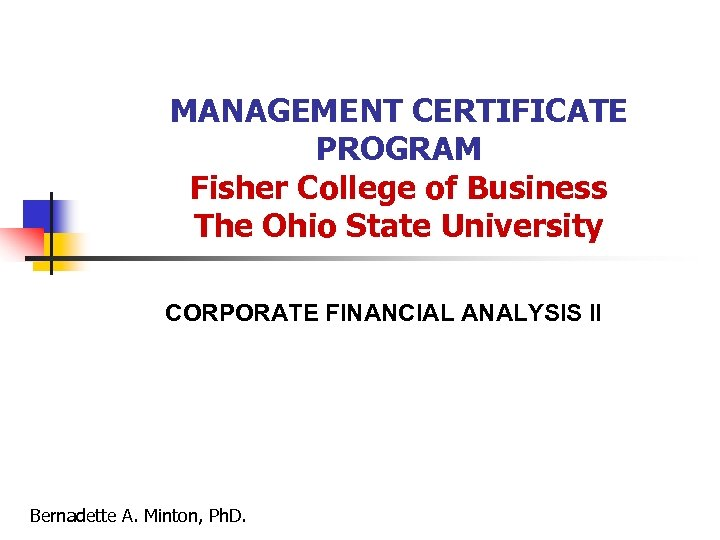MANAGEMENT CERTIFICATE PROGRAM Fisher College of Business The Ohio State University CORPORATE FINANCIAL ANALYSIS II Bernadette A. Minton, Ph. D.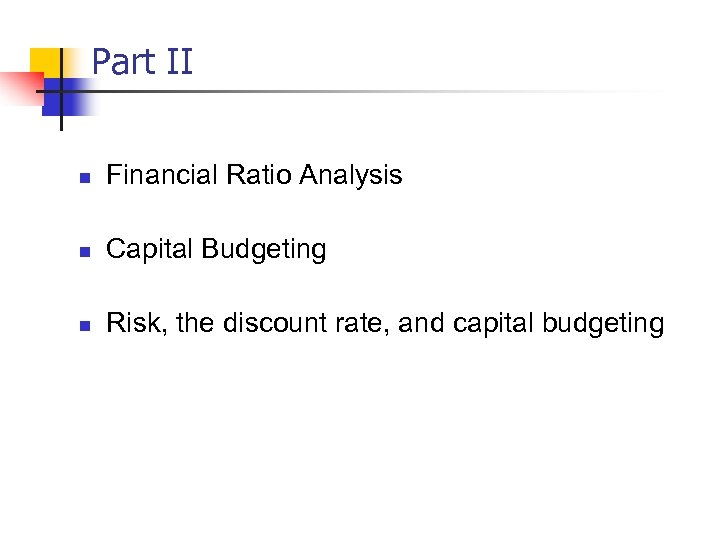Part II n Financial Ratio Analysis n Capital Budgeting n Risk, the discount rate, and capital budgeting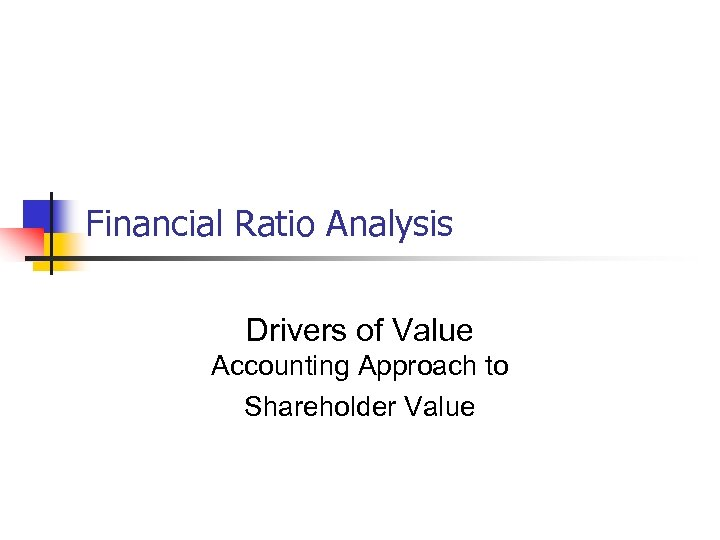Financial Ratio Analysis Drivers of Value Accounting Approach to Shareholder Value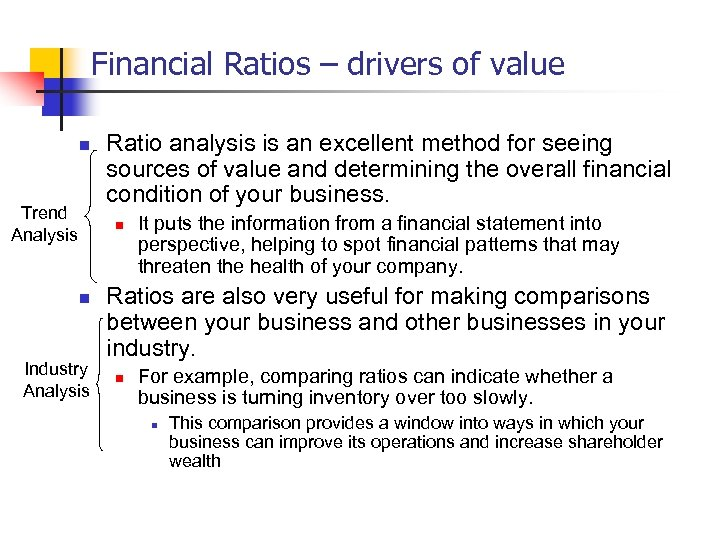Financial Ratios – drivers of value n Trend Analysis Ratio analysis is an excellent method for seeing sources of value and determining the overall financial condition of your business. n n Industry Analysis It puts the information from a financial statement into perspective, helping to spot financial patterns that may threaten the health of your company. Ratios are also very useful for making comparisons between your business and other businesses in your industry. n For example, comparing ratios can indicate whether a business is turning inventory over too slowly. n This comparison provides a window into ways in which your business can improve its operations and increase shareholder wealth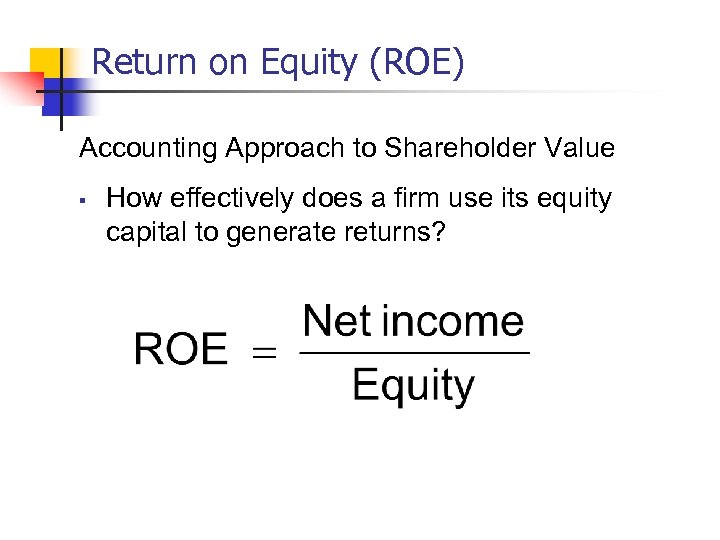Return on Equity (ROE) Accounting Approach to Shareholder Value § How effectively does a firm use its equity capital to generate returns?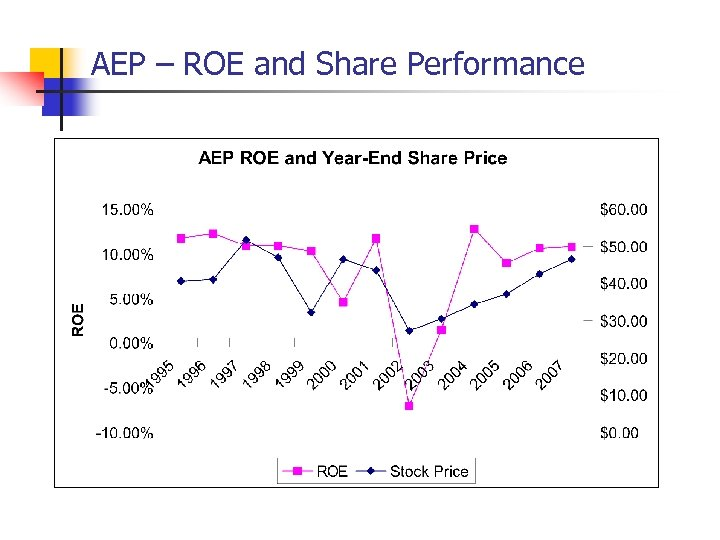AEP – ROE and Share Performance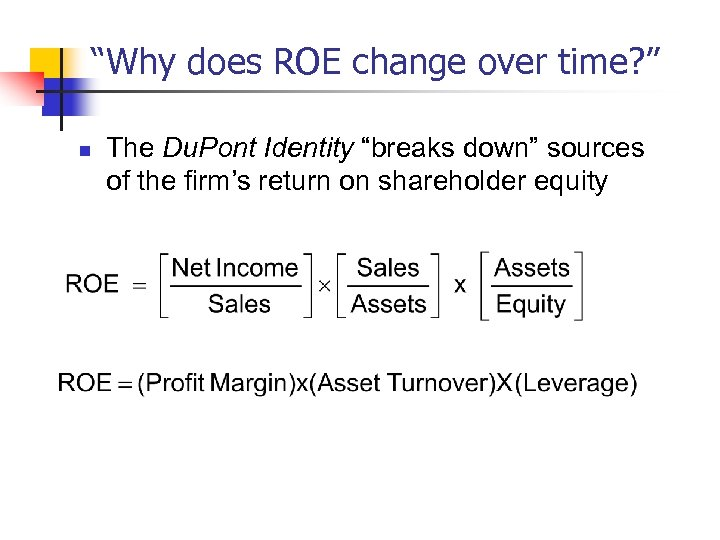“Why does ROE change over time? ” n The Du. Pont Identity “breaks down” sources of the firm’s return on shareholder equity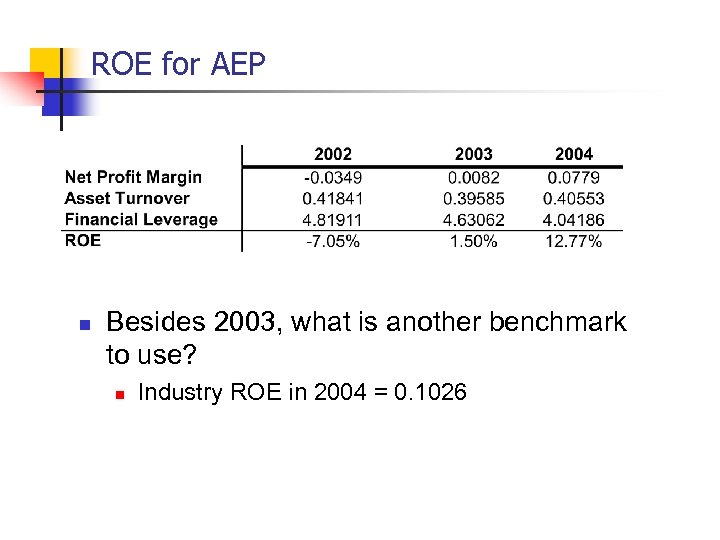ROE for AEP n Besides 2003, what is another benchmark to use? n Industry ROE in 2004 = 0. 1026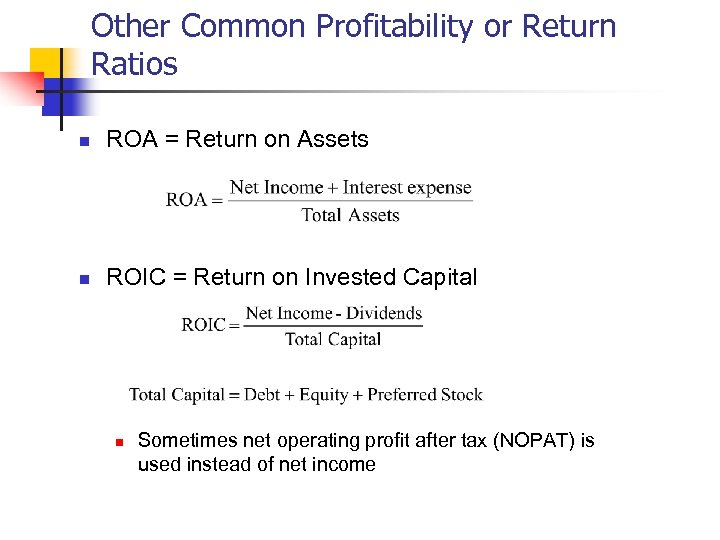Other Common Profitability or Return Ratios n ROA = Return on Assets n ROIC = Return on Invested Capital n Sometimes net operating profit after tax (NOPAT) is used instead of net income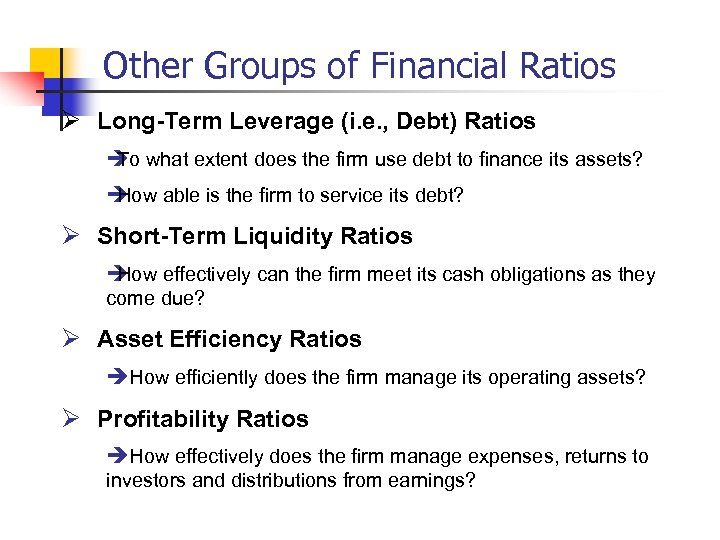Other Groups of Financial Ratios Ø Long-Term Leverage (i. e. , Debt) Ratios è what extent does the firm use debt to finance its assets? To è How able is the firm to service its debt? Ø Short-Term Liquidity Ratios è How effectively can the firm meet its cash obligations as they come due? Ø Asset Efficiency Ratios èHow efficiently does the firm manage its operating assets? Ø Profitability Ratios èHow effectively does the firm manage expenses, returns to investors and distributions from earnings?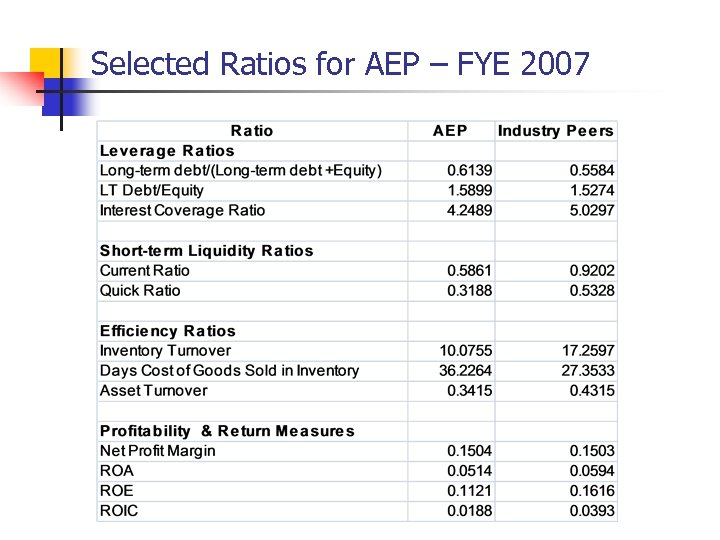Selected Ratios for AEP – FYE 2007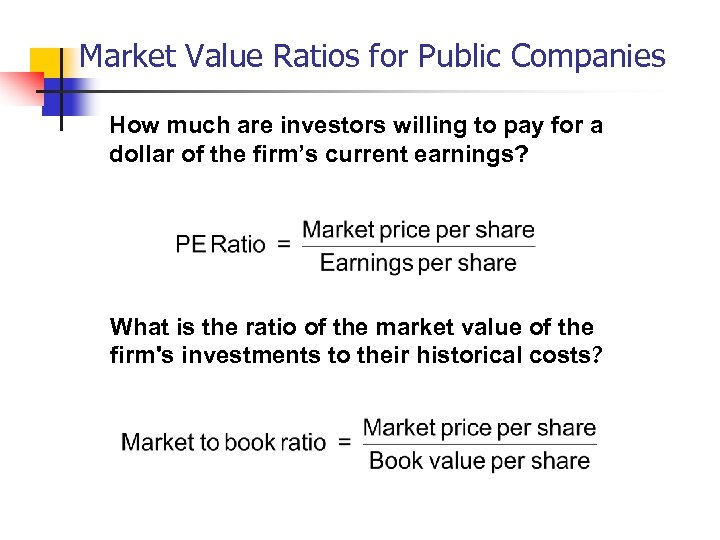Market Value Ratios for Public Companies How much are investors willing to pay for a dollar of the firm’s current earnings? What is the ratio of the market value of the firm's investments to their historical costs?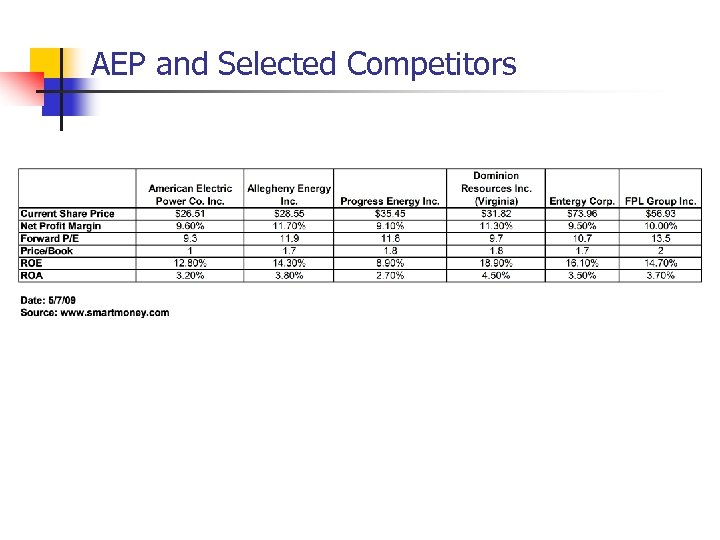AEP and Selected Competitors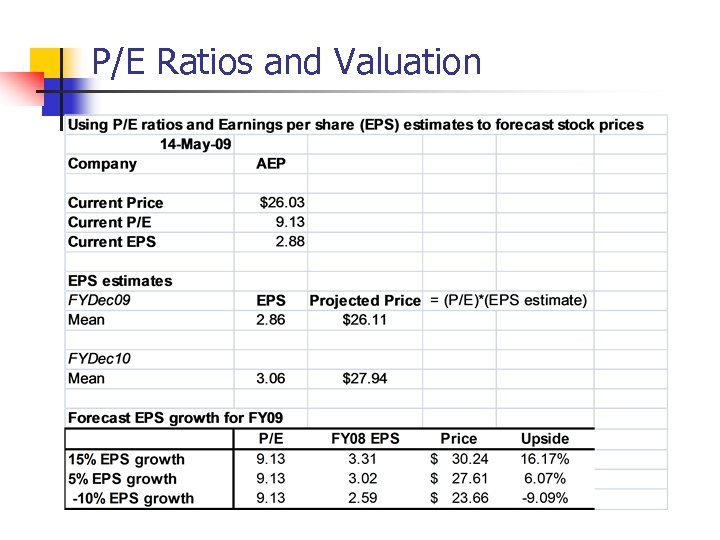P/E Ratios and Valuation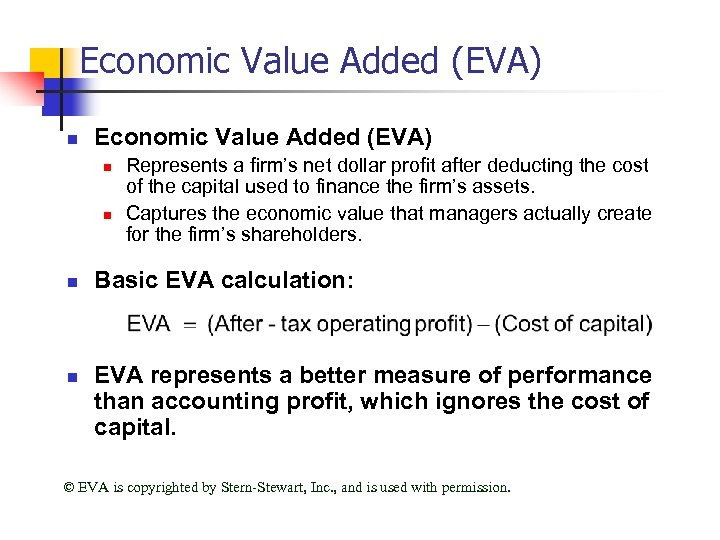Economic Value Added (EVA) n n n n Represents a firm’s net dollar profit after deducting the cost of the capital used to finance the firm’s assets. Captures the economic value that managers actually create for the firm’s shareholders. Basic EVA calculation: EVA represents a better measure of performance than accounting profit, which ignores the cost of capital. EVA is copyrighted by Stern-Stewart, Inc. , and is used with permission.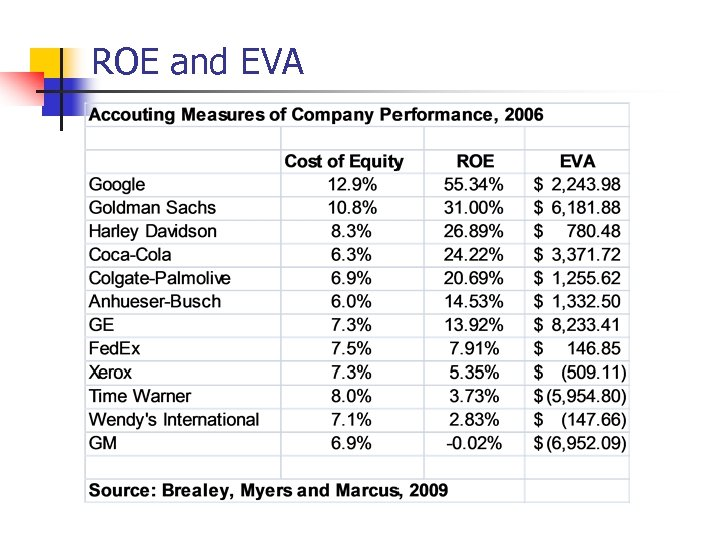ROE and EVA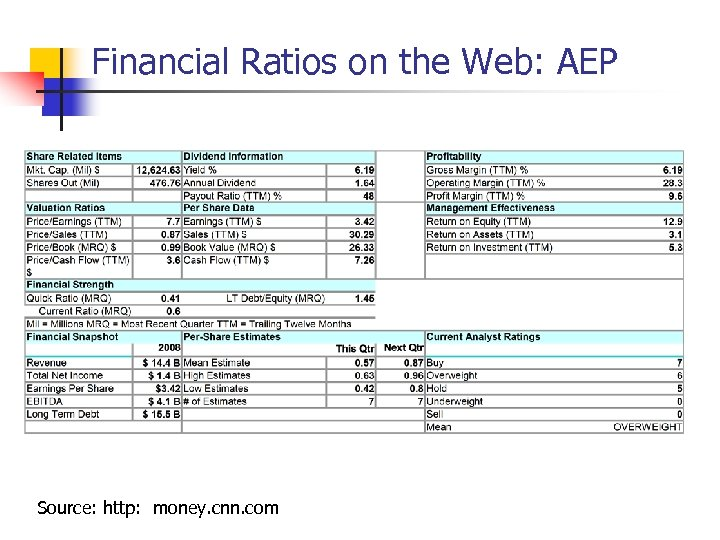Financial Ratios on the Web: AEP Source: http: money. cnn. com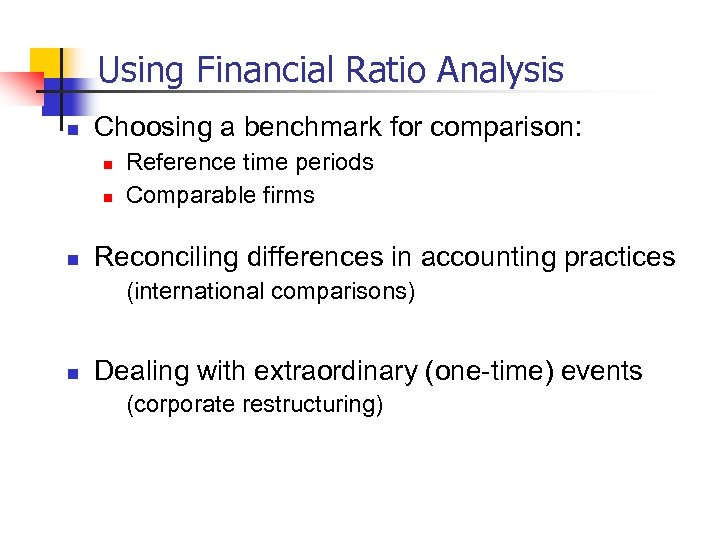Using Financial Ratio Analysis n Choosing a benchmark for comparison: n n n Reference time periods Comparable firms Reconciling differences in accounting practices (international comparisons) n Dealing with extraordinary (one-time) events (corporate restructuring)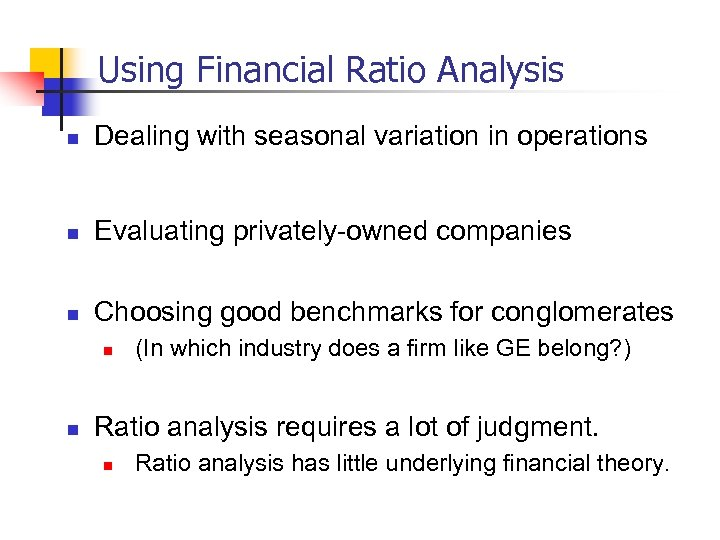Using Financial Ratio Analysis n Dealing with seasonal variation in operations n Evaluating privately-owned companies n Choosing good benchmarks for conglomerates n n (In which industry does a firm like GE belong? ) Ratio analysis requires a lot of judgment. n Ratio analysis has little underlying financial theory.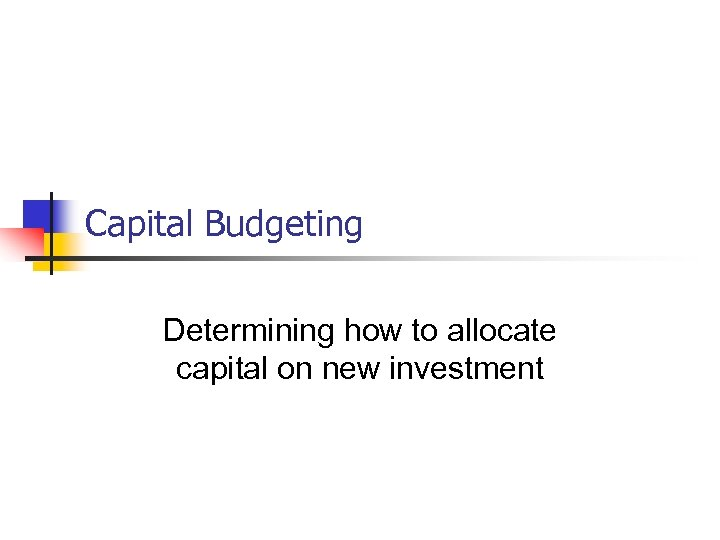Capital Budgeting Determining how to allocate capital on new investment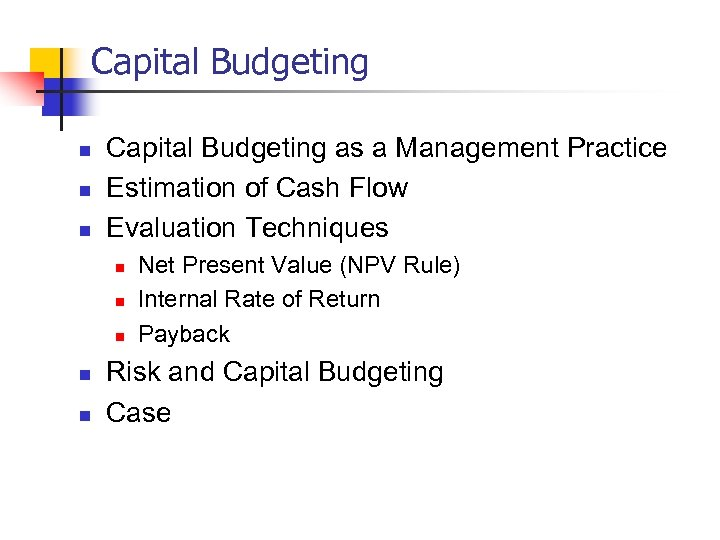Capital Budgeting n n n Capital Budgeting as a Management Practice Estimation of Cash Flow Evaluation Techniques n n n Net Present Value (NPV Rule) Internal Rate of Return Payback Risk and Capital Budgeting Case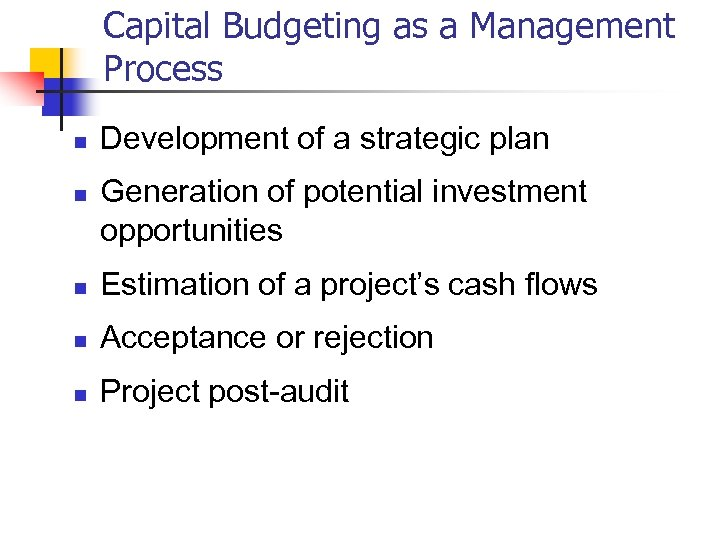Capital Budgeting as a Management Process n n Development of a strategic plan Generation of potential investment opportunities n Estimation of a project’s cash flows n Acceptance or rejection n Project post-audit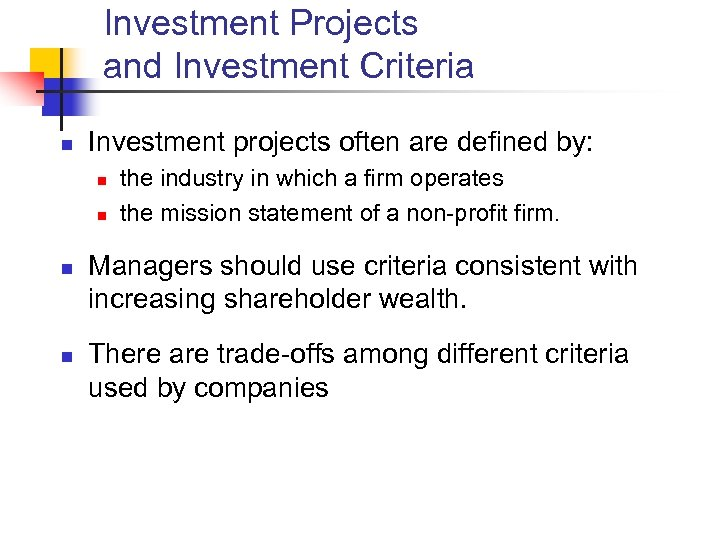Investment Projects and Investment Criteria n Investment projects often are defined by: n n the industry in which a firm operates the mission statement of a non-profit firm. Managers should use criteria consistent with increasing shareholder wealth. There are trade-offs among different criteria used by companies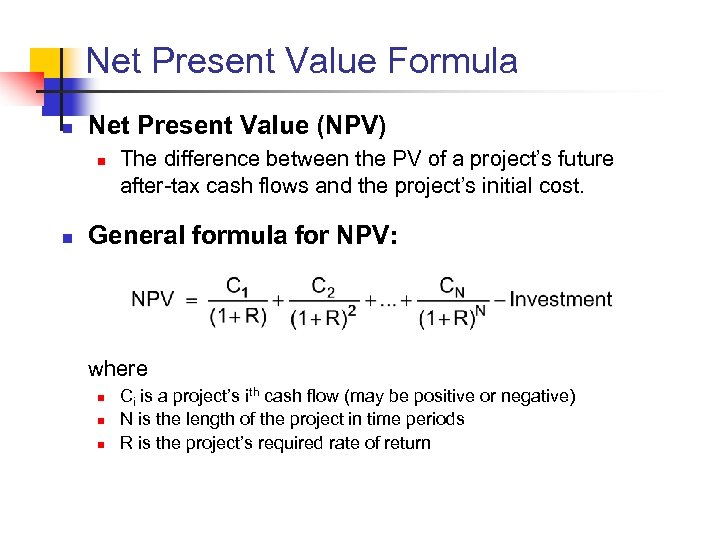Net Present Value Formula n Net Present Value (NPV) n n The difference between the PV of a project’s future after-tax cash flows and the project’s initial cost. General formula for NPV: where n n n Ci is a project’s ith cash flow (may be positive or negative) N is the length of the project in time periods R is the project’s required rate of return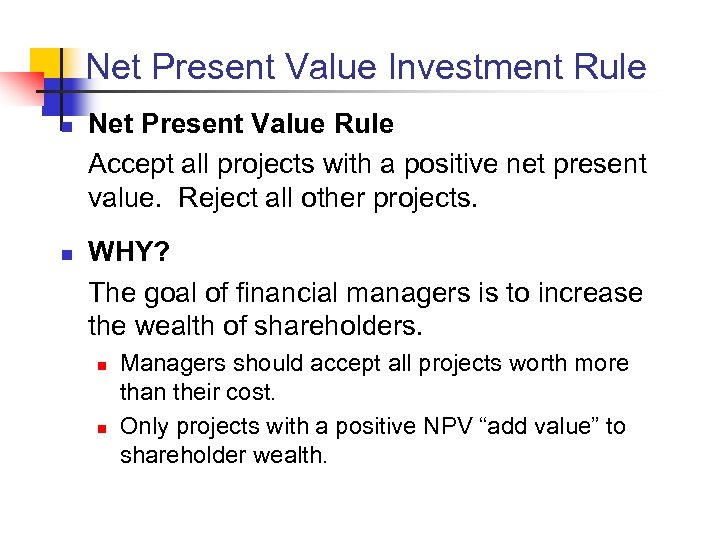Net Present Value Investment Rule n n Net Present Value Rule Accept all projects with a positive net present value. Reject all other projects. WHY? The goal of financial managers is to increase the wealth of shareholders. n n Managers should accept all projects worth more than their cost. Only projects with a positive NPV “add value” to shareholder wealth.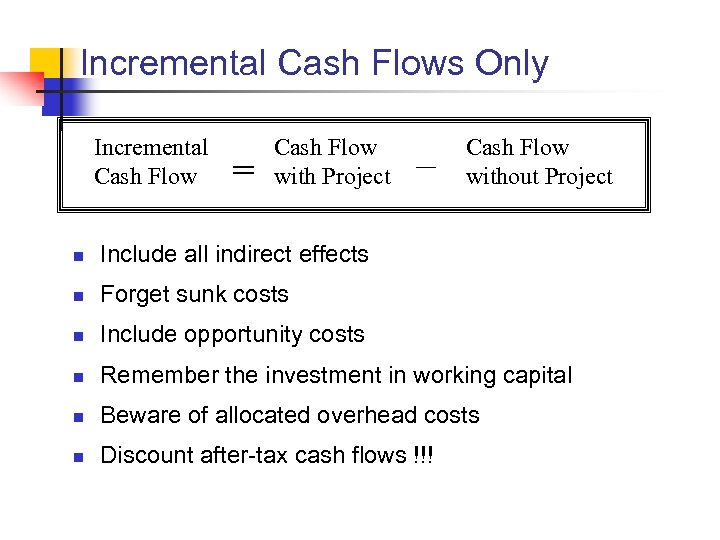Incremental Cash Flows Only Incremental Cash Flow = Cash Flow with Project – Cash Flow without Project n Include all indirect effects n Forget sunk costs n Include opportunity costs n Remember the investment in working capital n Beware of allocated overhead costs n Discount after-tax cash flows !!!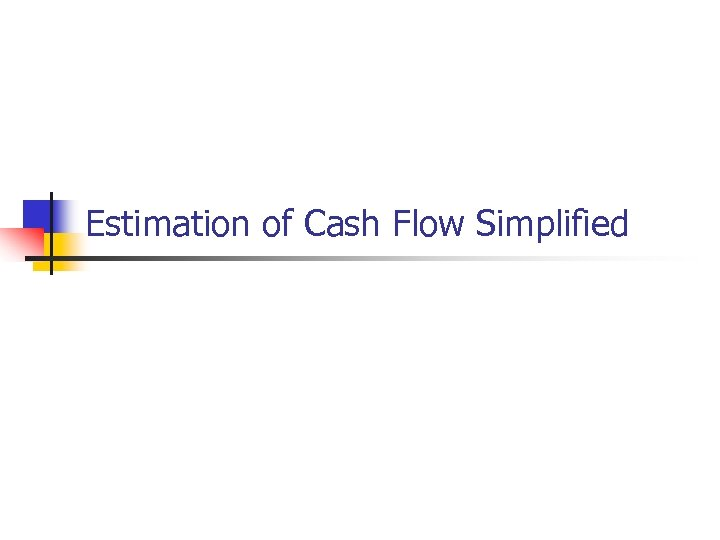Estimation of Cash Flow Simplified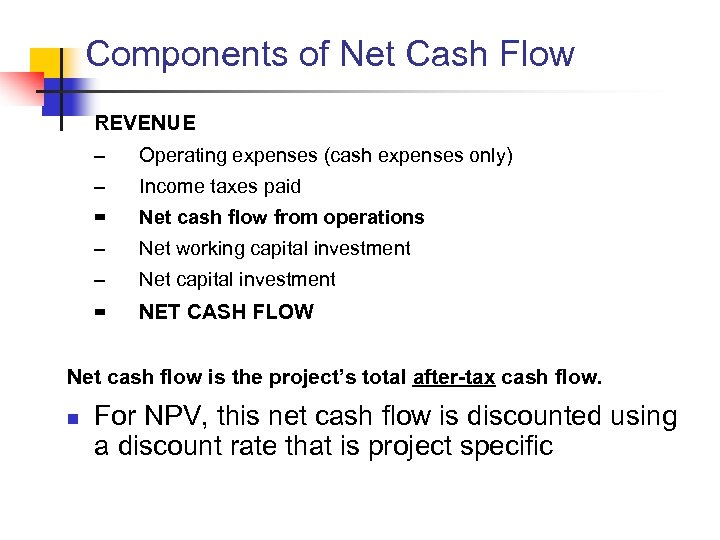Components of Net Cash Flow REVENUE – Operating expenses (cash expenses only) – Income taxes paid = Net cash flow from operations – Net working capital investment – Net capital investment = NET CASH FLOW Net cash flow is the project’s total after-tax cash flow. n For NPV, this net cash flow is discounted using a discount rate that is project specific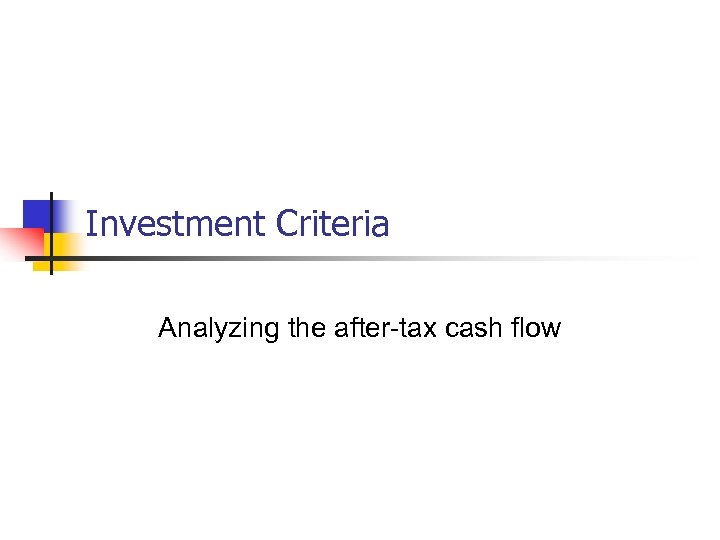Investment Criteria Analyzing the after-tax cash flow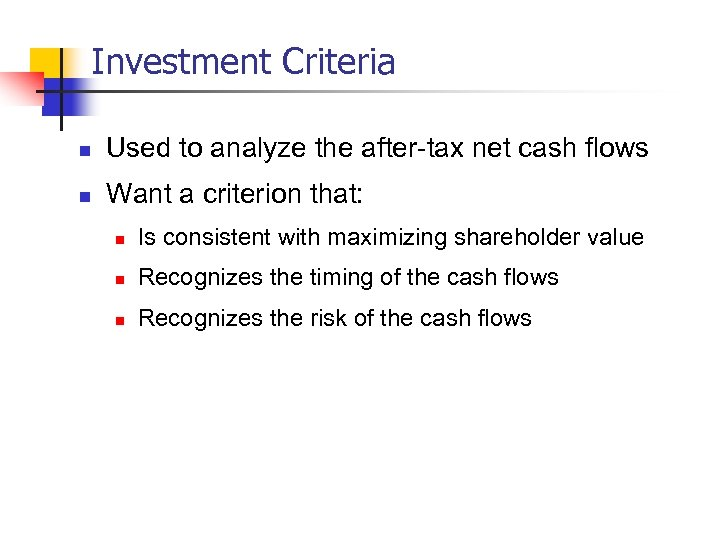Investment Criteria n Used to analyze the after-tax net cash flows n Want a criterion that: n Is consistent with maximizing shareholder value n Recognizes the timing of the cash flows n Recognizes the risk of the cash flows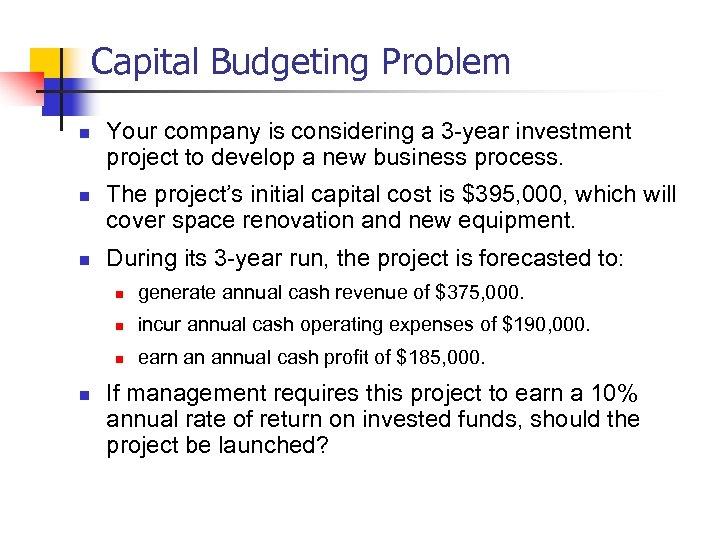Capital Budgeting Problem n n n Your company is considering a 3 -year investment project to develop a new business process. The project’s initial capital cost is \$395, 000, which will cover space renovation and new equipment. During its 3 -year run, the project is forecasted to: n n incur annual cash operating expenses of \$190, 000. n n generate annual cash revenue of \$375, 000. earn an annual cash profit of \$185, 000. If management requires this project to earn a 10% annual rate of return on invested funds, should the project be launched?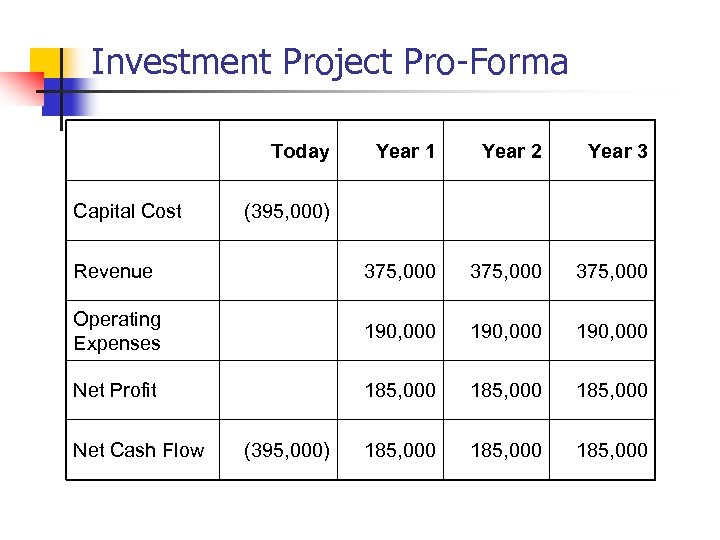Investment Project Pro-Forma Today Year 1 Year 2 Year 3 Revenue 375, 000 Operating Expenses 190, 000 Net Profit 185, 000 185, 000 Capital Cost Net Cash Flow (395, 000)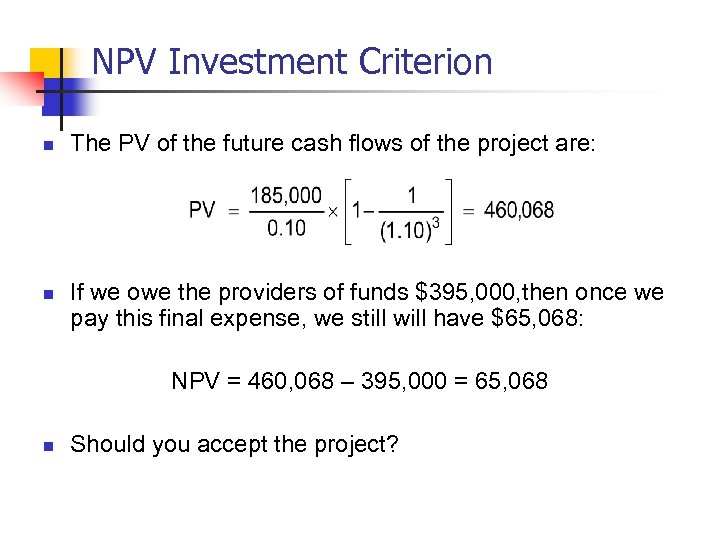NPV Investment Criterion n n The PV of the future cash flows of the project are: If we owe the providers of funds \$395, 000, then once we pay this final expense, we still will have \$65, 068: NPV = 460, 068 – 395, 000 = 65, 068 n Should you accept the project?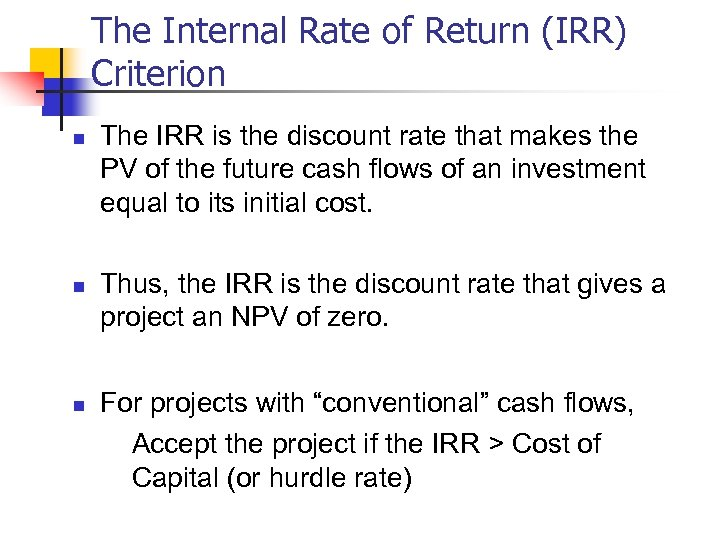The Internal Rate of Return (IRR) Criterion n The IRR is the discount rate that makes the PV of the future cash flows of an investment equal to its initial cost. Thus, the IRR is the discount rate that gives a project an NPV of zero. For projects with “conventional” cash flows, Accept the project if the IRR > Cost of Capital (or hurdle rate)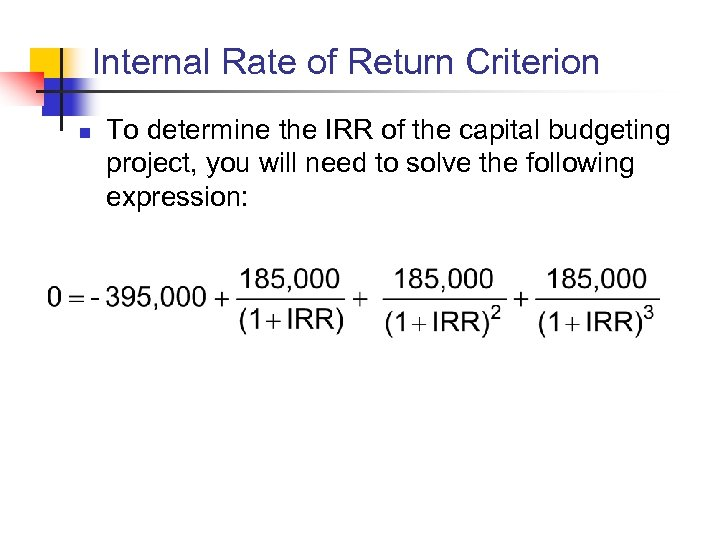Internal Rate of Return Criterion n To determine the IRR of the capital budgeting project, you will need to solve the following expression: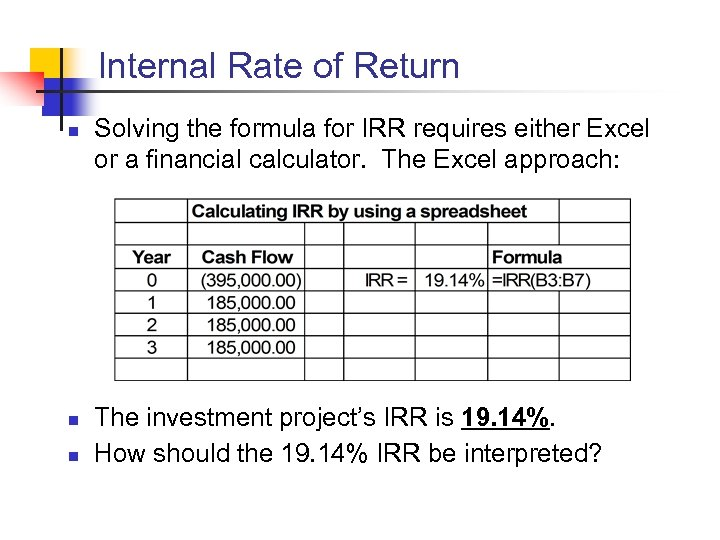Internal Rate of Return n Solving the formula for IRR requires either Excel or a financial calculator. The Excel approach: The investment project’s IRR is 19. 14%. How should the 19. 14% IRR be interpreted?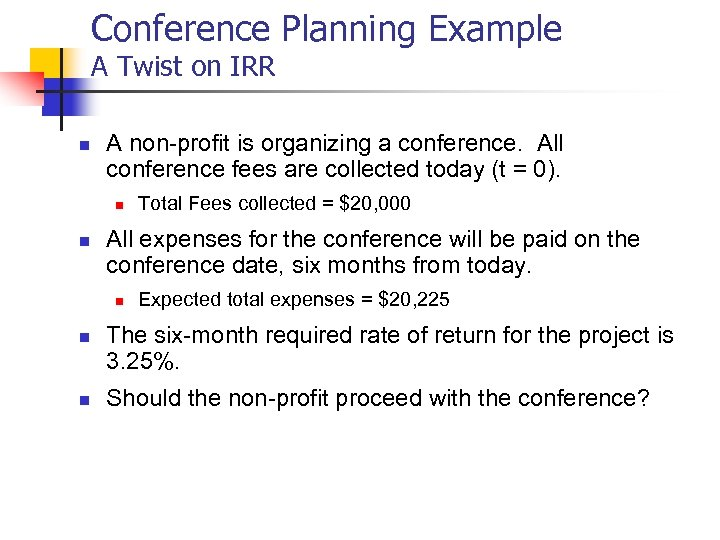Conference Planning Example A Twist on IRR n A non-profit is organizing a conference. All conference fees are collected today (t = 0). n n All expenses for the conference will be paid on the conference date, six months from today. n n n Total Fees collected = \$20, 000 Expected total expenses = \$20, 225 The six-month required rate of return for the project is 3. 25%. Should the non-profit proceed with the conference?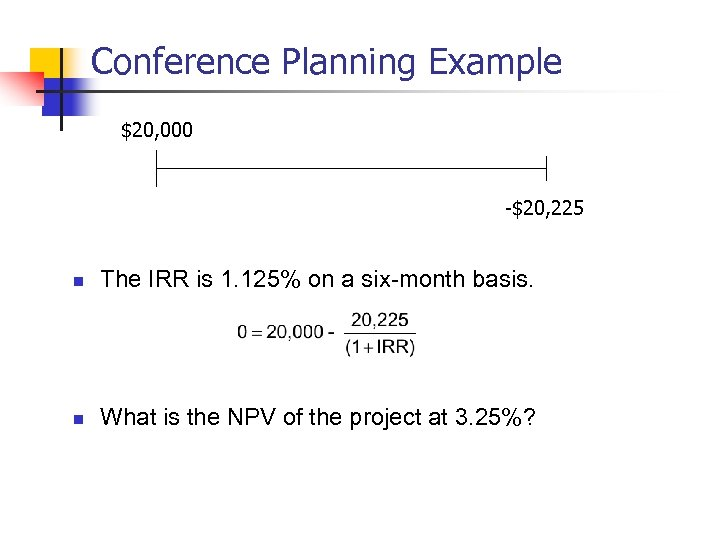Conference Planning Example \$20, 000 -\$20, 225 n The IRR is 1. 125% on a six-month basis. n What is the NPV of the project at 3. 25%?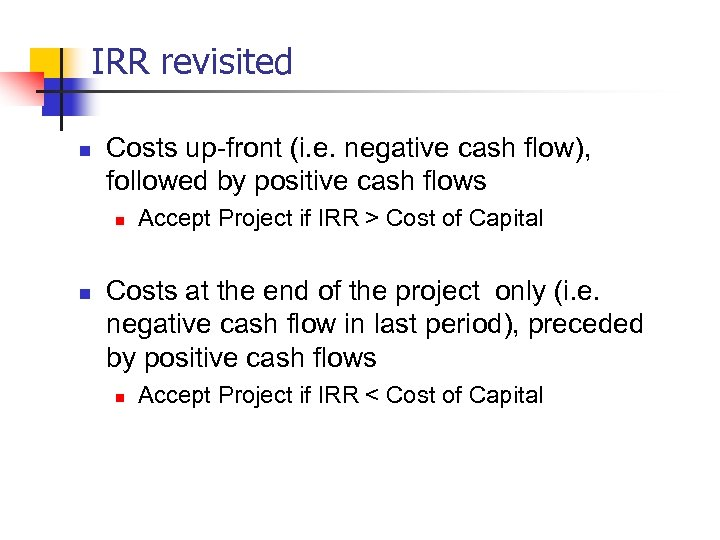IRR revisited n Costs up-front (i. e. negative cash flow), followed by positive cash flows n n Accept Project if IRR > Cost of Capital Costs at the end of the project only (i. e. negative cash flow in last period), preceded by positive cash flows n Accept Project if IRR < Cost of Capital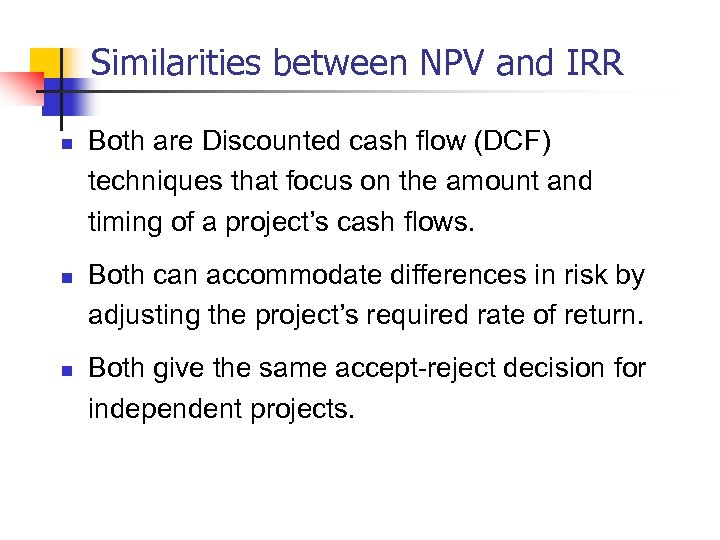Similarities between NPV and IRR n n n Both are Discounted cash flow (DCF) techniques that focus on the amount and timing of a project’s cash flows. Both can accommodate differences in risk by adjusting the project’s required rate of return. Both give the same accept-reject decision for independent projects.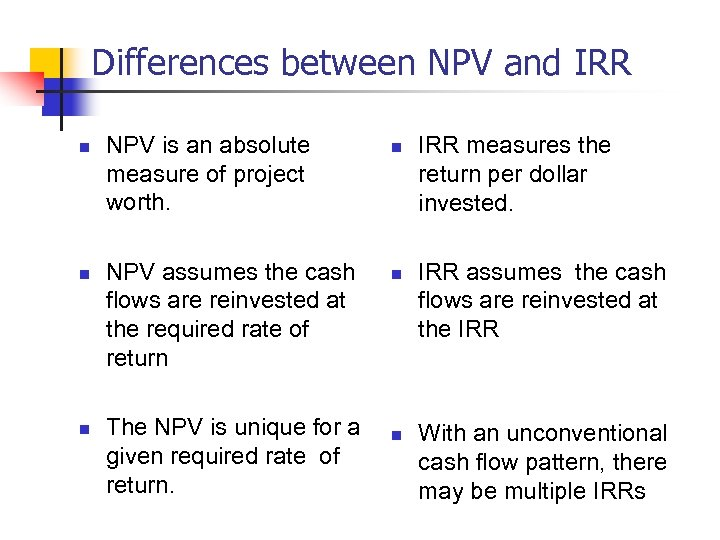Differences between NPV and IRR n n n NPV is an absolute measure of project worth. NPV assumes the cash flows are reinvested at the required rate of return The NPV is unique for a given required rate of return. n n n IRR measures the return per dollar invested. IRR assumes the cash flows are reinvested at the IRR With an unconventional cash flow pattern, there may be multiple IRRs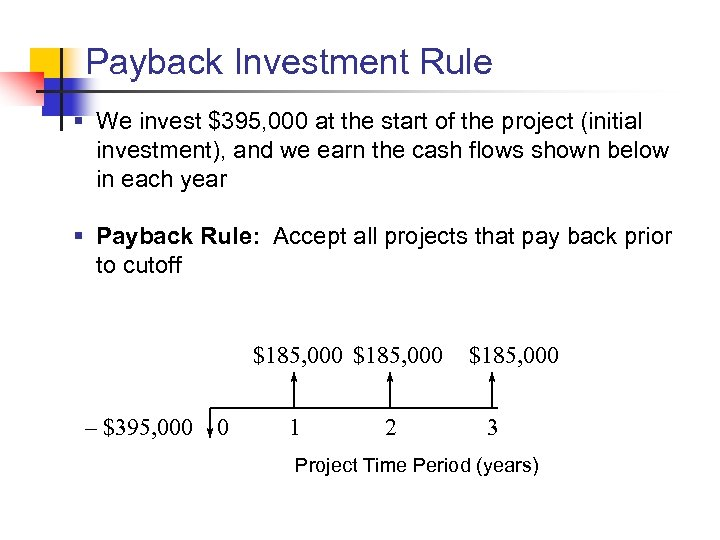Payback Investment Rule § We invest \$395, 000 at the start of the project (initial investment), and we earn the cash flows shown below in each year § Payback Rule: Accept all projects that pay back prior to cutoff \$185, 000 – \$395, 000 0 1 2 \$185, 000 3 Project Time Period (years)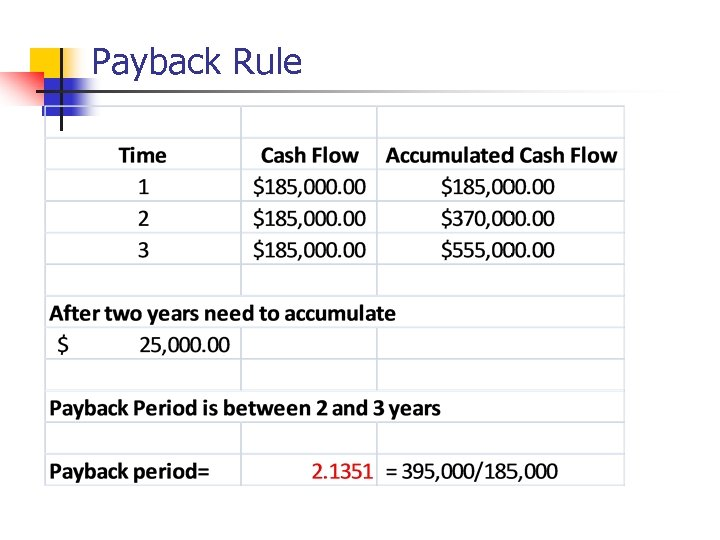Payback Rule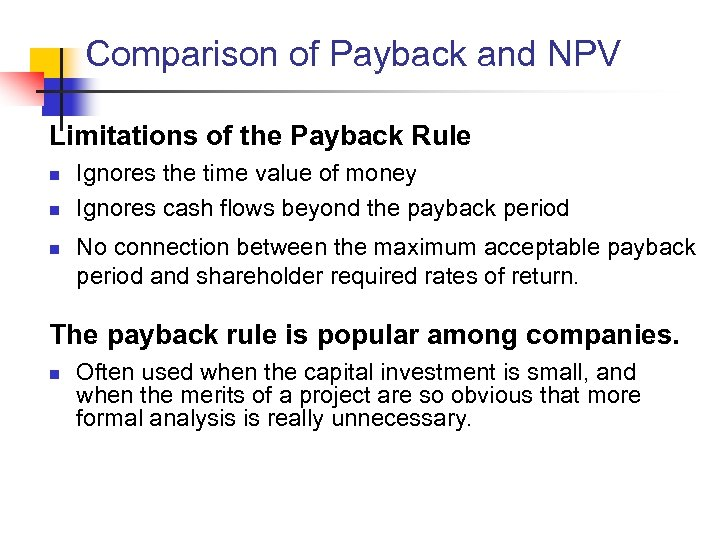Comparison of Payback and NPV Limitations of the Payback Rule n n n Ignores the time value of money Ignores cash flows beyond the payback period No connection between the maximum acceptable payback period and shareholder required rates of return. The payback rule is popular among companies. n Often used when the capital investment is small, and when the merits of a project are so obvious that more formal analysis is really unnecessary.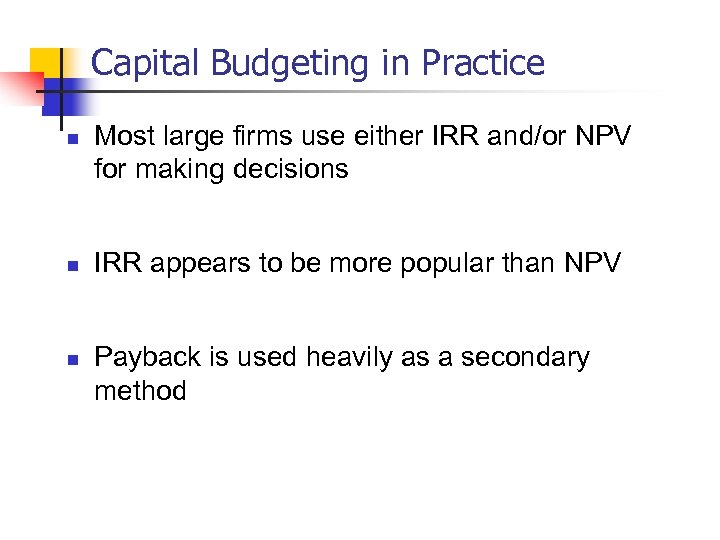Capital Budgeting in Practice n n n Most large firms use either IRR and/or NPV for making decisions IRR appears to be more popular than NPV Payback is used heavily as a secondary method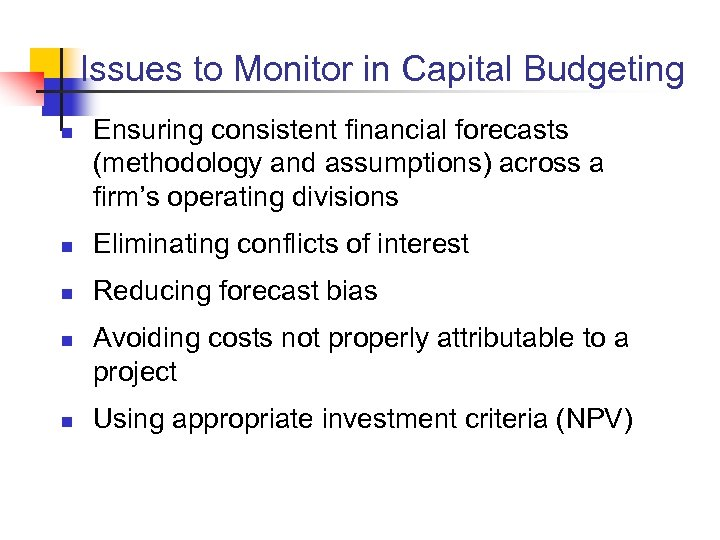Issues to Monitor in Capital Budgeting n Ensuring consistent financial forecasts (methodology and assumptions) across a firm’s operating divisions n Eliminating conflicts of interest n Reducing forecast bias n n Avoiding costs not properly attributable to a project Using appropriate investment criteria (NPV)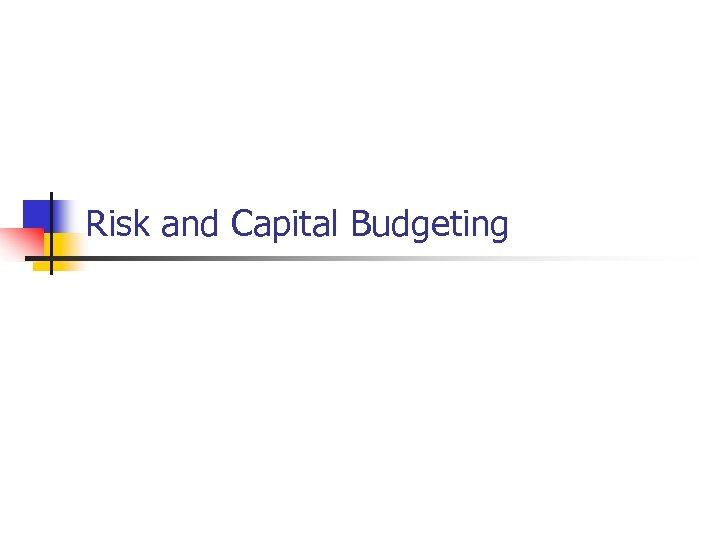Risk and Capital Budgeting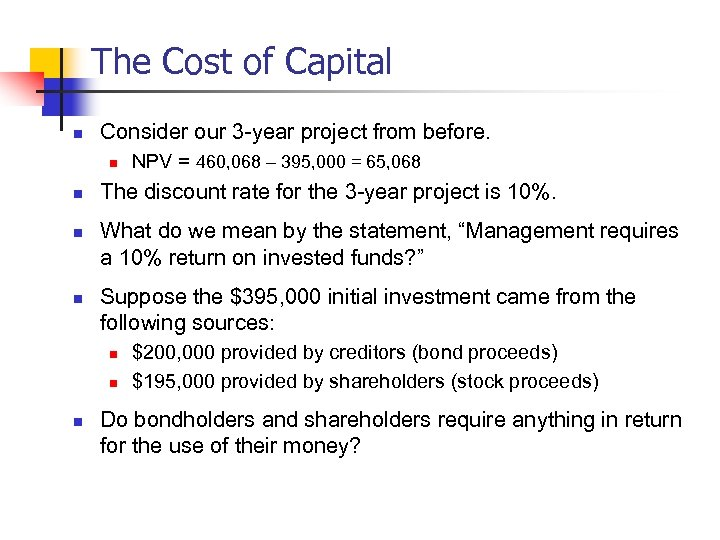The Cost of Capital n Consider our 3 -year project from before. n n The discount rate for the 3 -year project is 10%. What do we mean by the statement, “Management requires a 10% return on invested funds? ” Suppose the \$395, 000 initial investment came from the following sources: n n n NPV = 460, 068 – 395, 000 = 65, 068 \$200, 000 provided by creditors (bond proceeds) \$195, 000 provided by shareholders (stock proceeds) Do bondholders and shareholders require anything in return for the use of their money?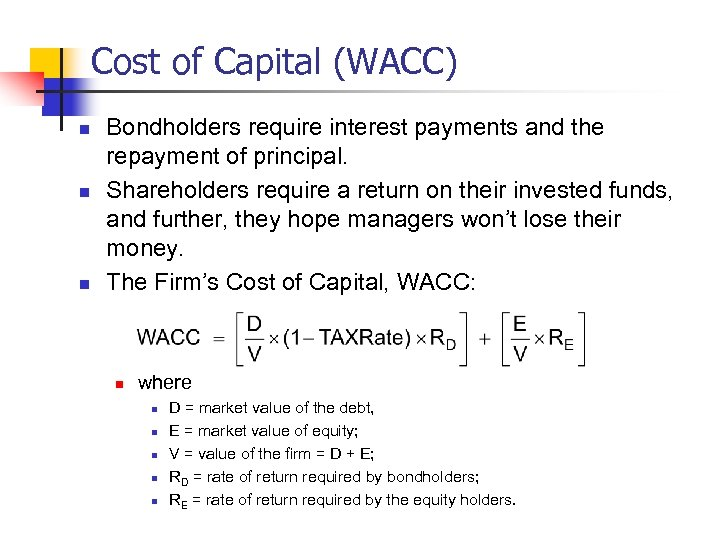Cost of Capital (WACC) n n n Bondholders require interest payments and the repayment of principal. Shareholders require a return on their invested funds, and further, they hope managers won’t lose their money. The Firm’s Cost of Capital, WACC: n where n n n D = market value of the debt, E = market value of equity; V = value of the firm = D + E; RD = rate of return required by bondholders; RE = rate of return required by the equity holders.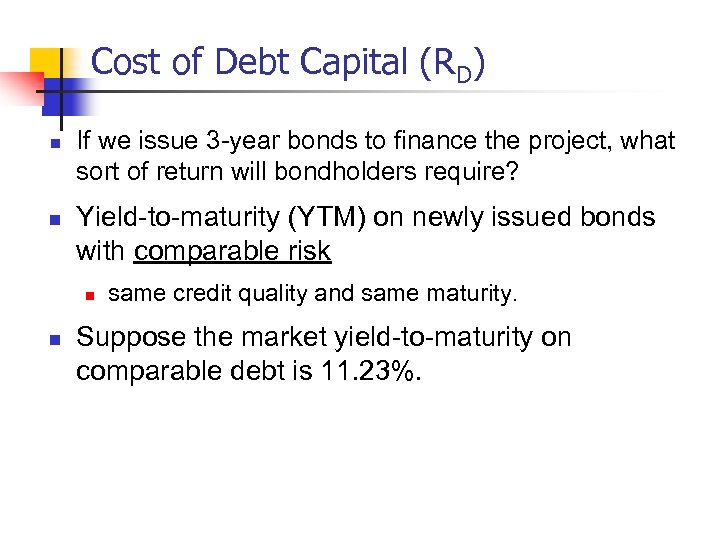Cost of Debt Capital (RD) n n If we issue 3 -year bonds to finance the project, what sort of return will bondholders require? Yield-to-maturity (YTM) on newly issued bonds with comparable risk n n same credit quality and same maturity. Suppose the market yield-to-maturity on comparable debt is 11. 23%.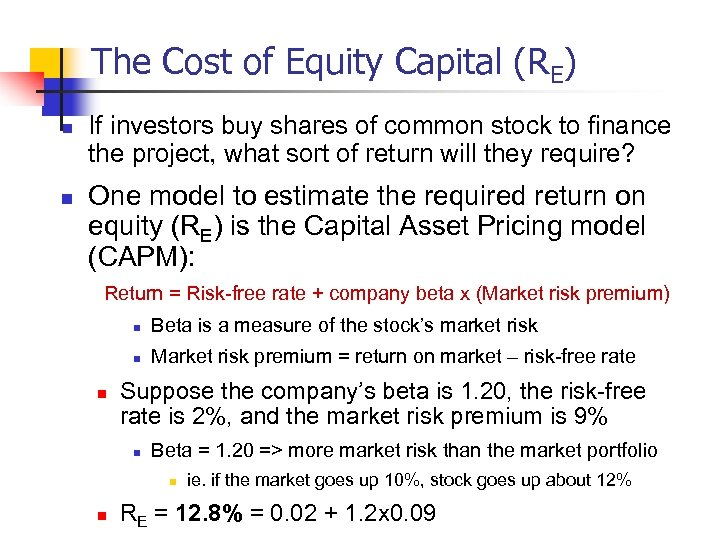The Cost of Equity Capital (RE) n n If investors buy shares of common stock to finance the project, what sort of return will they require? One model to estimate the required return on equity (RE) is the Capital Asset Pricing model (CAPM): Return = Risk-free rate + company beta x (Market risk premium) n n n Beta is a measure of the stock’s market risk Market risk premium = return on market – risk-free rate Suppose the company’s beta is 1. 20, the risk-free rate is 2%, and the market risk premium is 9% n Beta = 1. 20 => more market risk than the market portfolio n n ie. if the market goes up 10%, stock goes up about 12% RE = 12. 8% = 0. 02 + 1. 2 x 0. 09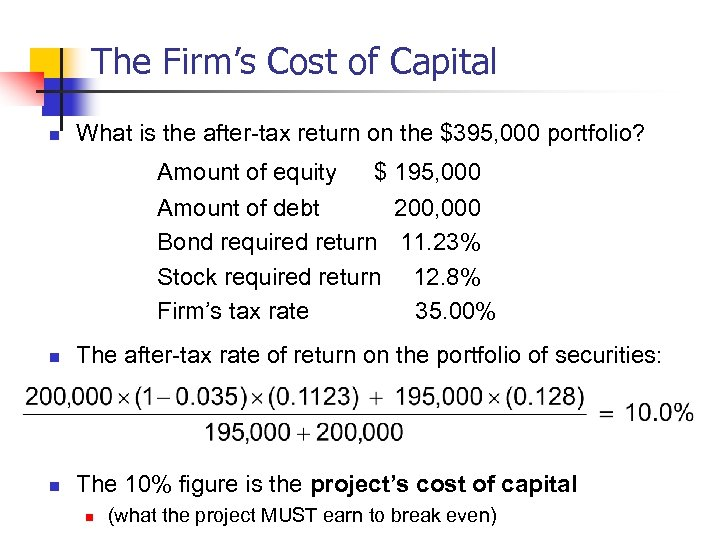The Firm’s Cost of Capital n What is the after-tax return on the \$395, 000 portfolio? Amount of equity \$ 195, 000 Amount of debt 200, 000 Bond required return 11. 23% Stock required return 12. 8% Firm’s tax rate 35. 00% n The after-tax rate of return on the portfolio of securities: n The 10% figure is the project’s cost of capital n (what the project MUST earn to break even)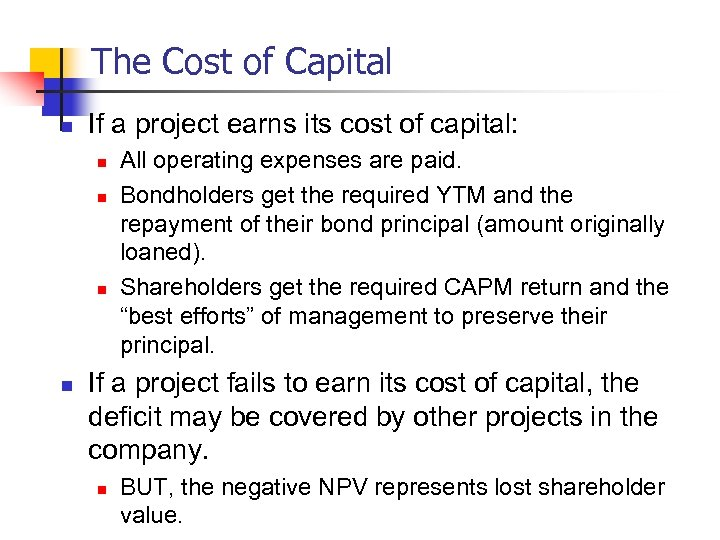The Cost of Capital n If a project earns its cost of capital: n n All operating expenses are paid. Bondholders get the required YTM and the repayment of their bond principal (amount originally loaned). Shareholders get the required CAPM return and the “best efforts” of management to preserve their principal. If a project fails to earn its cost of capital, the deficit may be covered by other projects in the company. n BUT, the negative NPV represents lost shareholder value.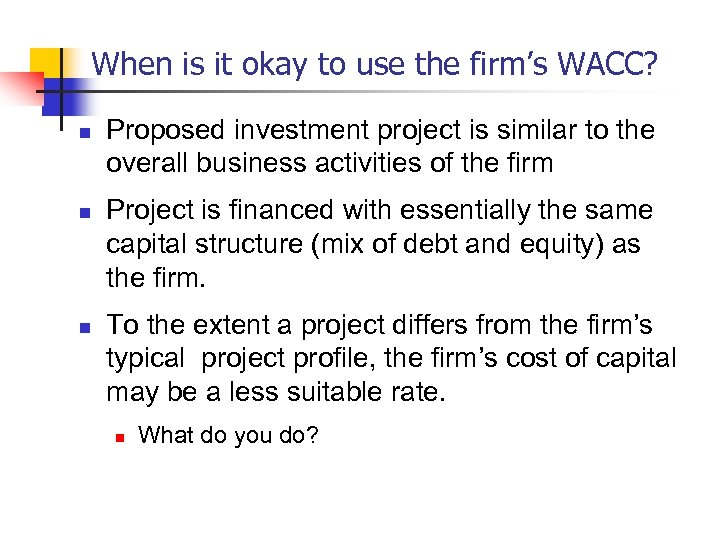When is it okay to use the firm’s WACC? n n n Proposed investment project is similar to the overall business activities of the firm Project is financed with essentially the same capital structure (mix of debt and equity) as the firm. To the extent a project differs from the firm’s typical project profile, the firm’s cost of capital may be a less suitable rate. n What do you do?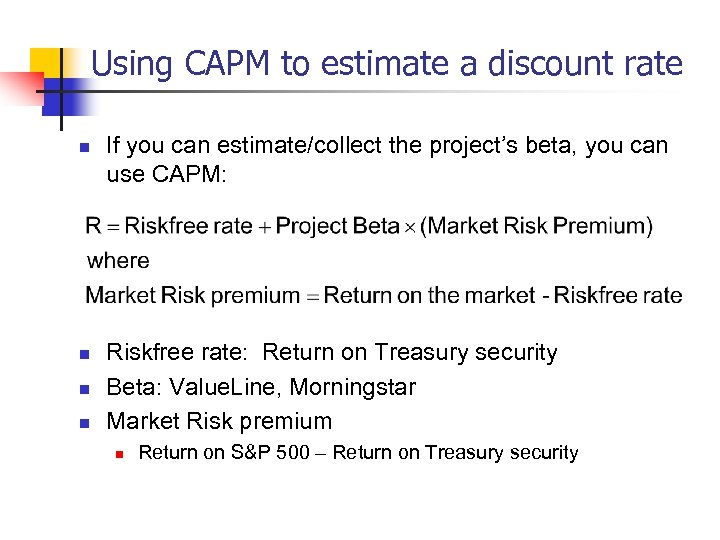Using CAPM to estimate a discount rate n n If you can estimate/collect the project’s beta, you can use CAPM: Riskfree rate: Return on Treasury security Beta: Value. Line, Morningstar Market Risk premium n Return on S&P 500 – Return on Treasury security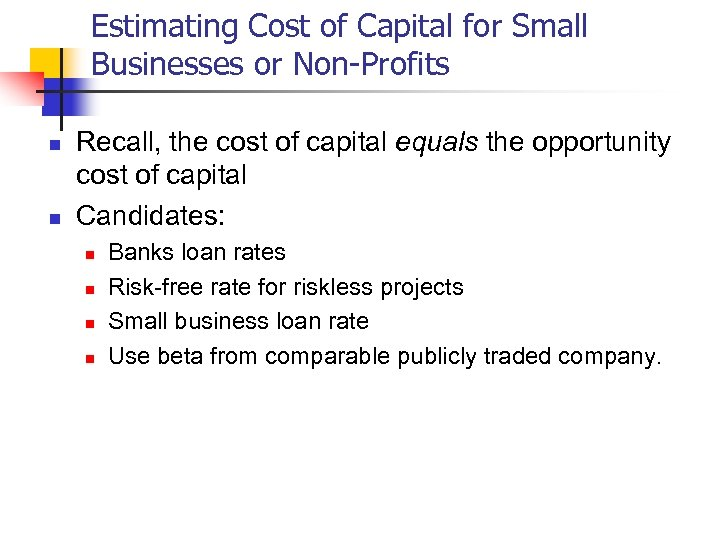Estimating Cost of Capital for Small Businesses or Non-Profits n n Recall, the cost of capital equals the opportunity cost of capital Candidates: n n Banks loan rates Risk-free rate for riskless projects Small business loan rate Use beta from comparable publicly traded company.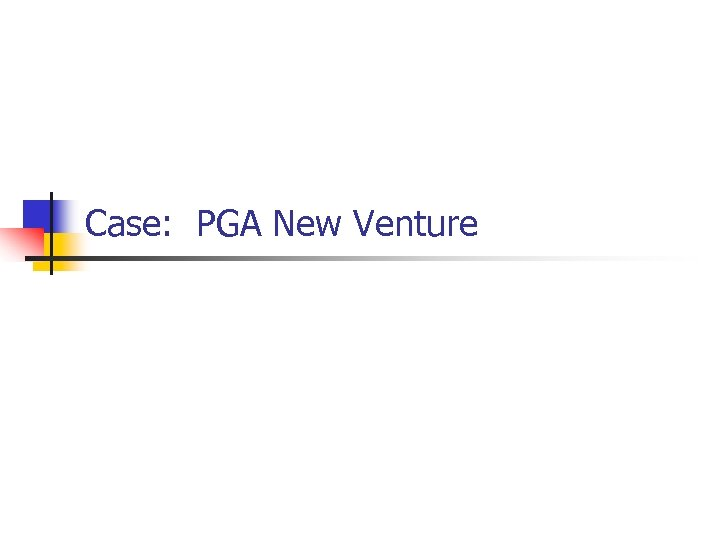Case: PGA New Venture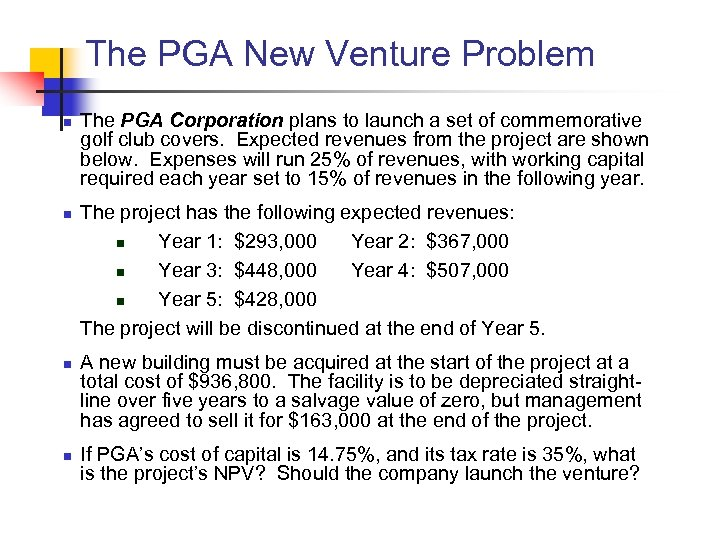The PGA New Venture Problem n n The PGA Corporation plans to launch a set of commemorative golf club covers. Expected revenues from the project are shown below. Expenses will run 25% of revenues, with working capital required each year set to 15% of revenues in the following year. The project has the following expected revenues: n Year 1: \$293, 000 Year 2: \$367, 000 n Year 3: \$448, 000 Year 4: \$507, 000 n Year 5: \$428, 000 The project will be discontinued at the end of Year 5. A new building must be acquired at the start of the project at a total cost of \$936, 800. The facility is to be depreciated straightline over five years to a salvage value of zero, but management has agreed to sell it for \$163, 000 at the end of the project. If PGA’s cost of capital is 14. 75%, and its tax rate is 35%, what is the project’s NPV? Should the company launch the venture?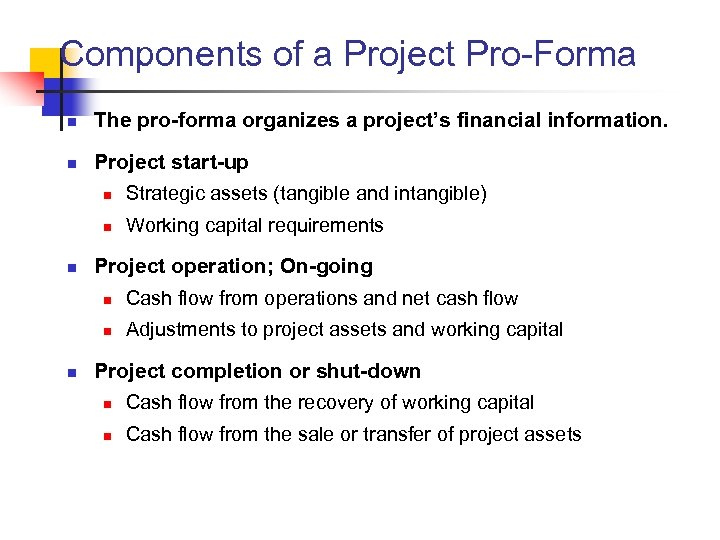Components of a Project Pro-Forma n The pro-forma organizes a project’s financial information. n Project start-up n n n Strategic assets (tangible and intangible) Working capital requirements Project operation; On-going n n n Cash flow from operations and net cash flow Adjustments to project assets and working capital Project completion or shut-down n Cash flow from the recovery of working capital n Cash flow from the sale or transfer of project assets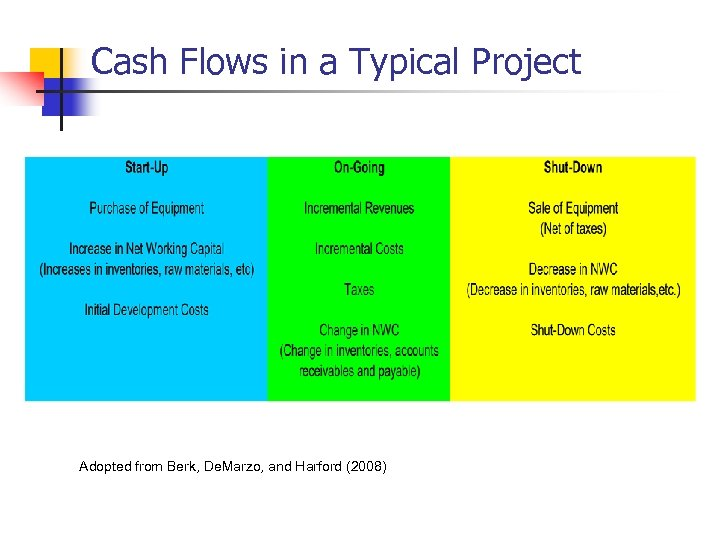Cash Flows in a Typical Project Adopted from Berk, De. Marzo, and Harford (2008)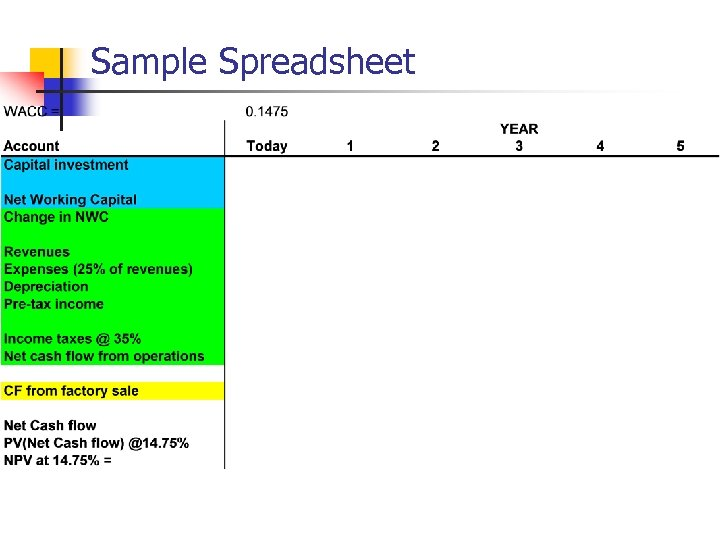Sample Spreadsheet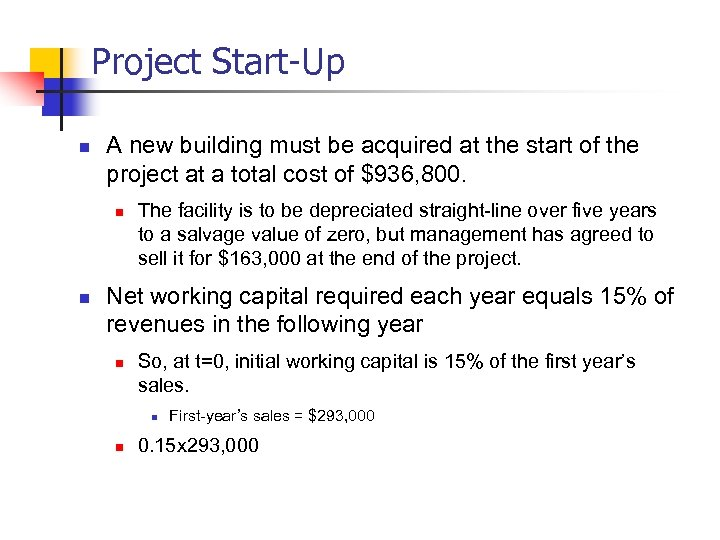Project Start-Up n A new building must be acquired at the start of the project at a total cost of \$936, 800. n n The facility is to be depreciated straight-line over five years to a salvage value of zero, but management has agreed to sell it for \$163, 000 at the end of the project. Net working capital required each year equals 15% of revenues in the following year n So, at t=0, initial working capital is 15% of the first year’s sales. n n First-year’s sales = \$293, 000 0. 15 x 293, 000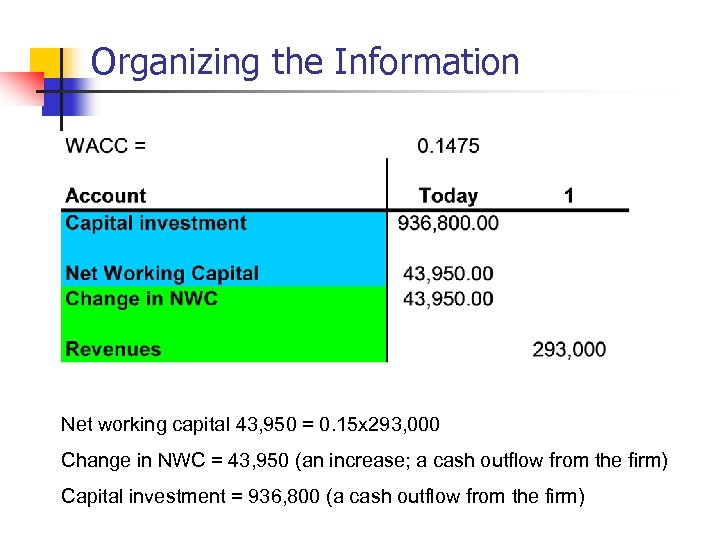Organizing the Information Net working capital 43, 950 = 0. 15 x 293, 000 Change in NWC = 43, 950 (an increase; a cash outflow from the firm) Capital investment = 936, 800 (a cash outflow from the firm)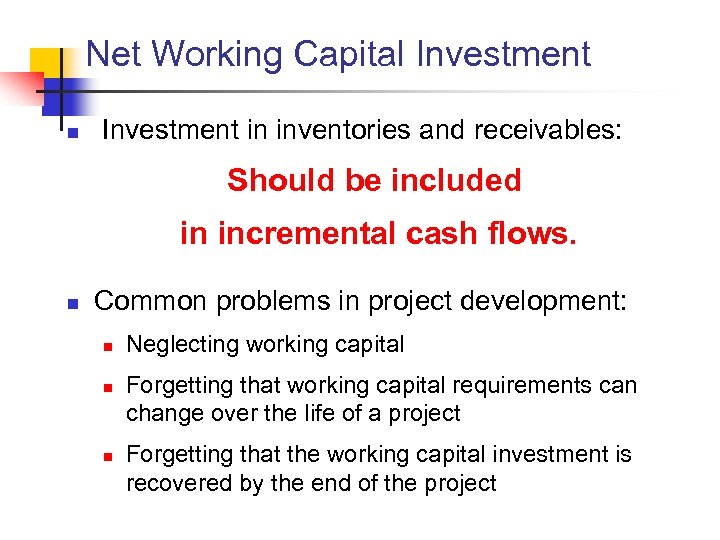Net Working Capital Investment n Investment in inventories and receivables: Should be included in incremental cash flows. n Common problems in project development: n n n Neglecting working capital Forgetting that working capital requirements can change over the life of a project Forgetting that the working capital investment is recovered by the end of the project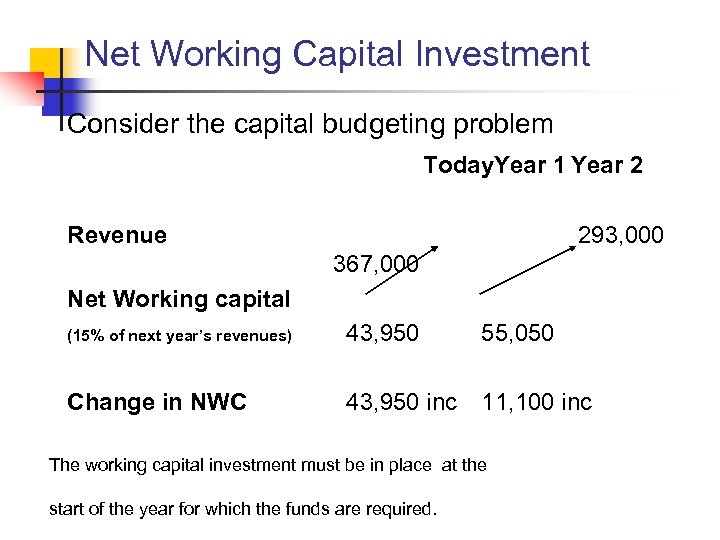Net Working Capital Investment Consider the capital budgeting problem Today. Year 1 Year 2 Revenue 293, 000 367, 000 Net Working capital (15% of next year’s revenues) 43, 950 55, 050 Change in NWC 43, 950 inc 11, 100 inc The working capital investment must be in place at the start of the year for which the funds are required.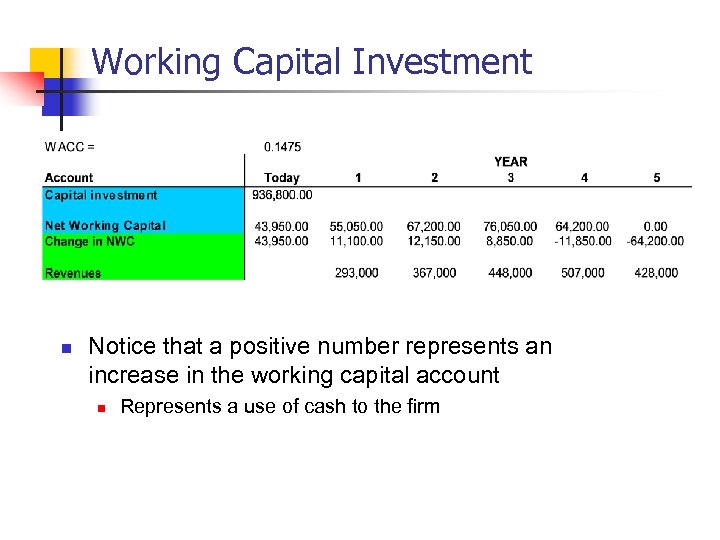Working Capital Investment n Notice that a positive number represents an increase in the working capital account n Represents a use of cash to the firm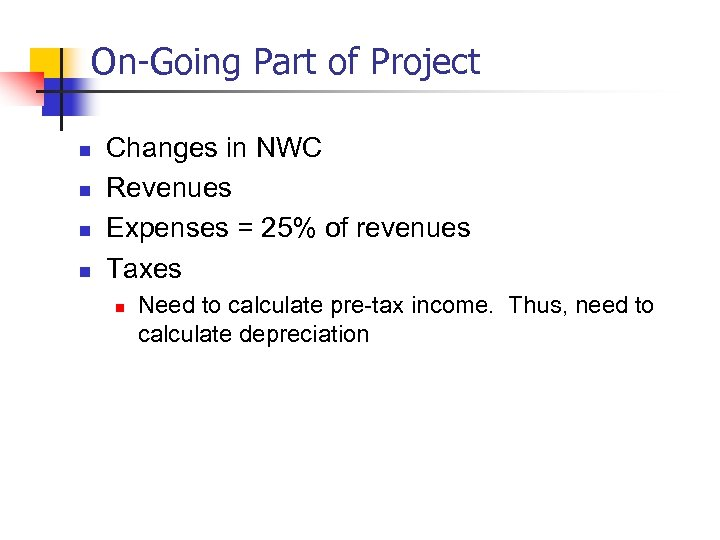On-Going Part of Project n n Changes in NWC Revenues Expenses = 25% of revenues Taxes n Need to calculate pre-tax income. Thus, need to calculate depreciation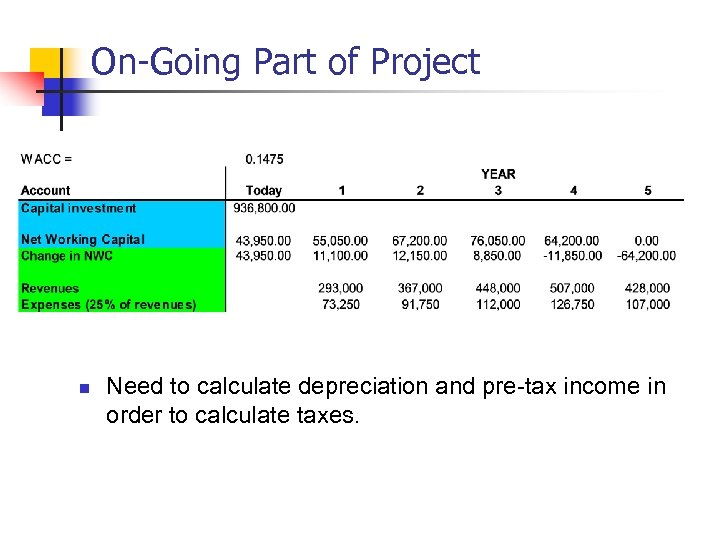On-Going Part of Project n Need to calculate depreciation and pre-tax income in order to calculate taxes.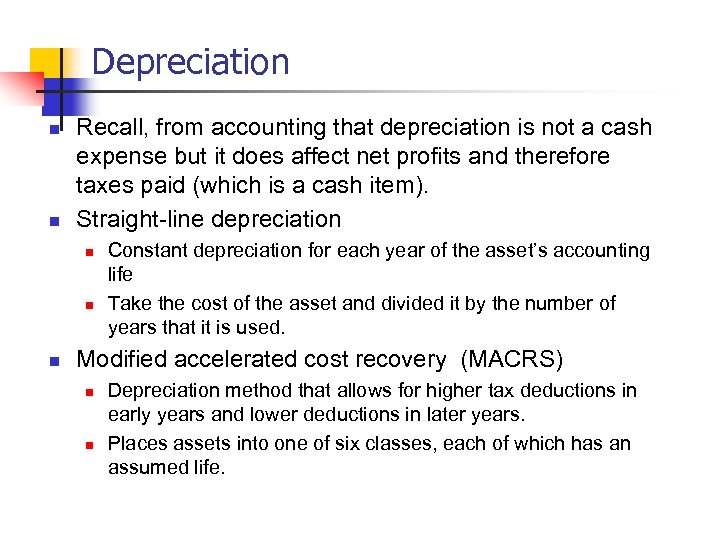Depreciation n n Recall, from accounting that depreciation is not a cash expense but it does affect net profits and therefore taxes paid (which is a cash item). Straight-line depreciation n Constant depreciation for each year of the asset’s accounting life Take the cost of the asset and divided it by the number of years that it is used. Modified accelerated cost recovery (MACRS) n n Depreciation method that allows for higher tax deductions in early years and lower deductions in later years. Places assets into one of six classes, each of which has an assumed life.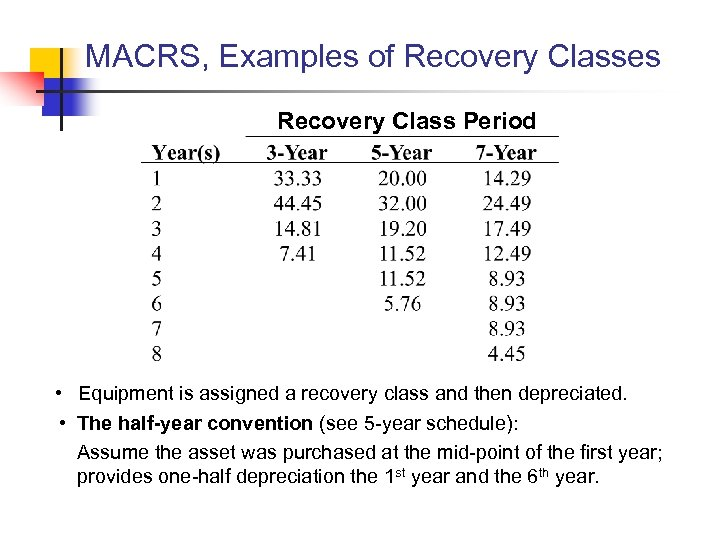MACRS, Examples of Recovery Classes Recovery Class Period • Equipment is assigned a recovery class and then depreciated. • The half-year convention (see 5 -year schedule): Assume the asset was purchased at the mid-point of the first year; provides one-half depreciation the 1 st year and the 6 th year.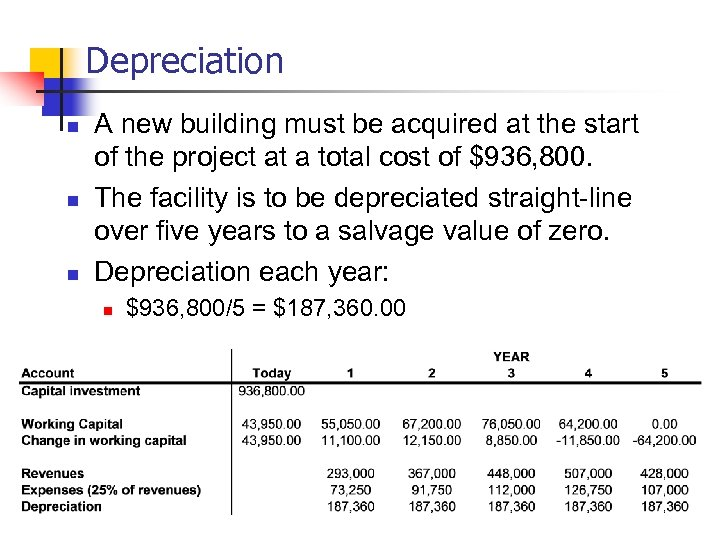Depreciation n A new building must be acquired at the start of the project at a total cost of \$936, 800. The facility is to be depreciated straight-line over five years to a salvage value of zero. Depreciation each year: n \$936, 800/5 = \$187, 360. 00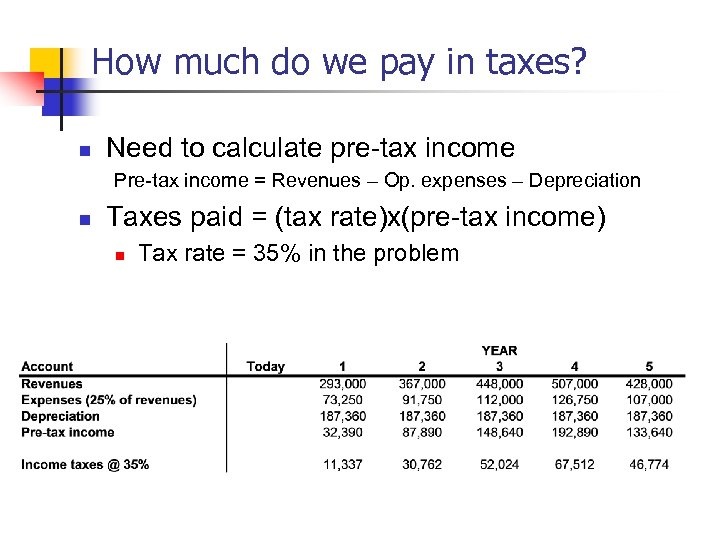How much do we pay in taxes? n Need to calculate pre-tax income Pre-tax income = Revenues – Op. expenses – Depreciation n Taxes paid = (tax rate)x(pre-tax income) n Tax rate = 35% in the problem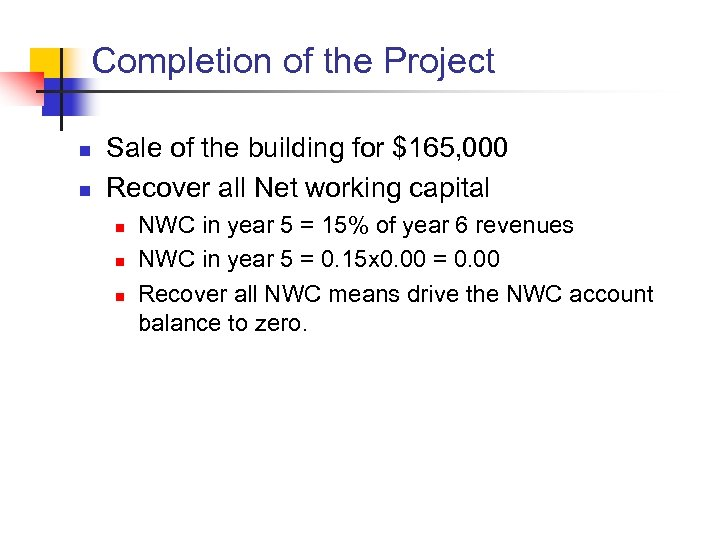Completion of the Project n n Sale of the building for \$165, 000 Recover all Net working capital n n n NWC in year 5 = 15% of year 6 revenues NWC in year 5 = 0. 15 x 0. 00 = 0. 00 Recover all NWC means drive the NWC account balance to zero.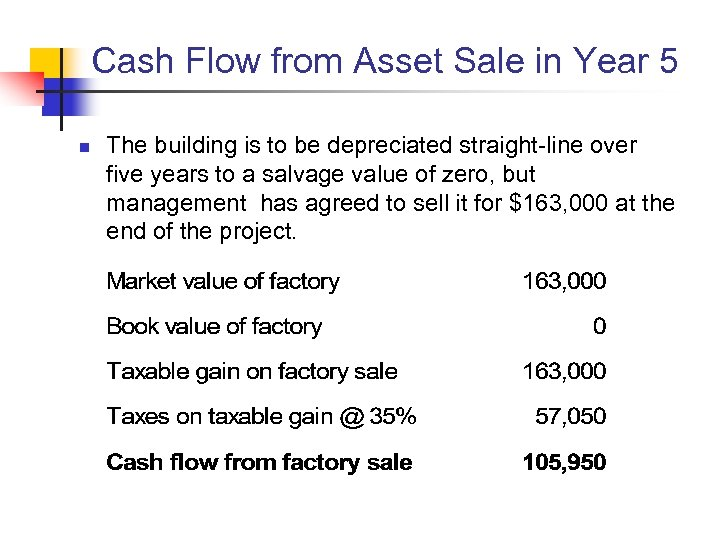Cash Flow from Asset Sale in Year 5 n The building is to be depreciated straight-line over five years to a salvage value of zero, but management has agreed to sell it for \$163, 000 at the end of the project.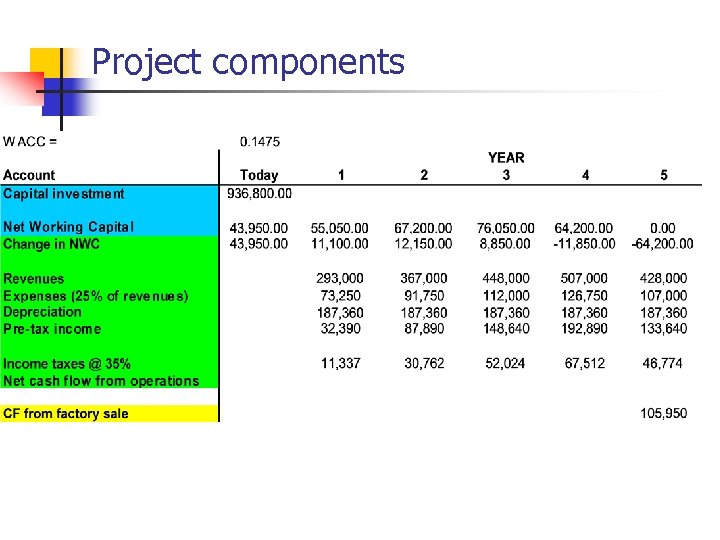Project components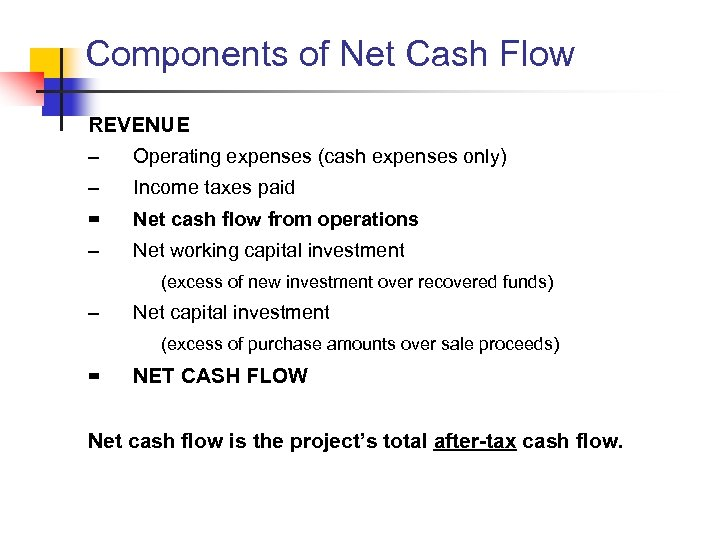Components of Net Cash Flow REVENUE – Operating expenses (cash expenses only) – Income taxes paid = Net cash flow from operations – Net working capital investment (excess of new investment over recovered funds) – Net capital investment (excess of purchase amounts over sale proceeds) = NET CASH FLOW Net cash flow is the project’s total after-tax cash flow.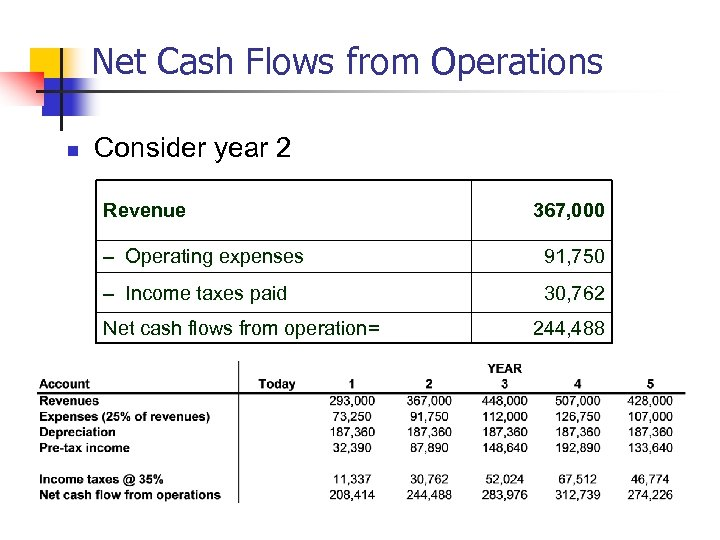Net Cash Flows from Operations n Consider year 2 Revenue 367, 000 – Operating expenses 91, 750 – Income taxes paid 30, 762 Net cash flows from operation= 244, 488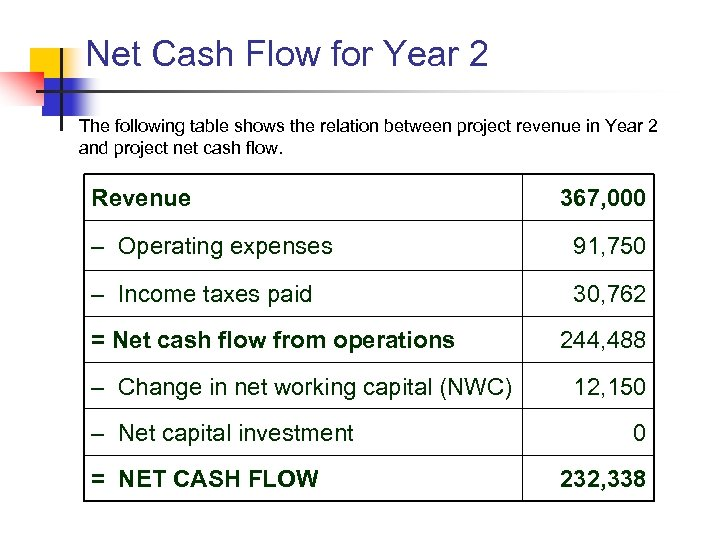Net Cash Flow for Year 2 The following table shows the relation between project revenue in Year 2 and project net cash flow. Revenue 367, 000 – Operating expenses 91, 750 – Income taxes paid 30, 762 = Net cash flow from operations – Change in net working capital (NWC) – Net capital investment = NET CASH FLOW 244, 488 12, 150 0 232, 338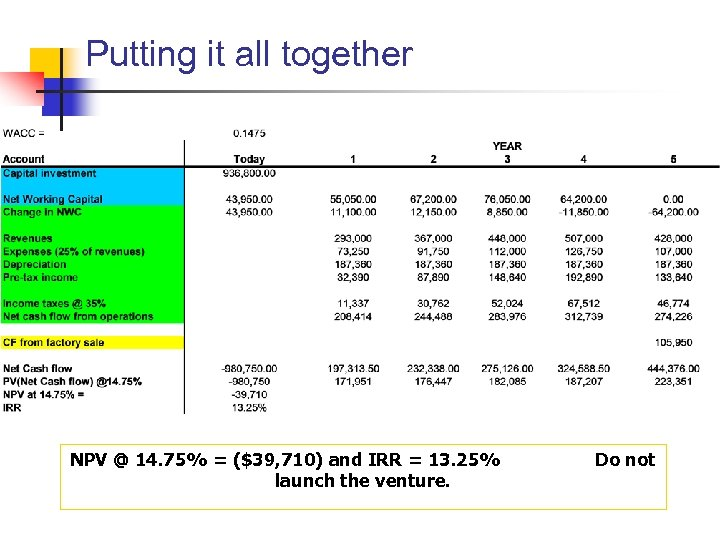Putting it all together NPV @ 14. 75% = (\$39, 710) and IRR = 13. 25% launch the venture. Do not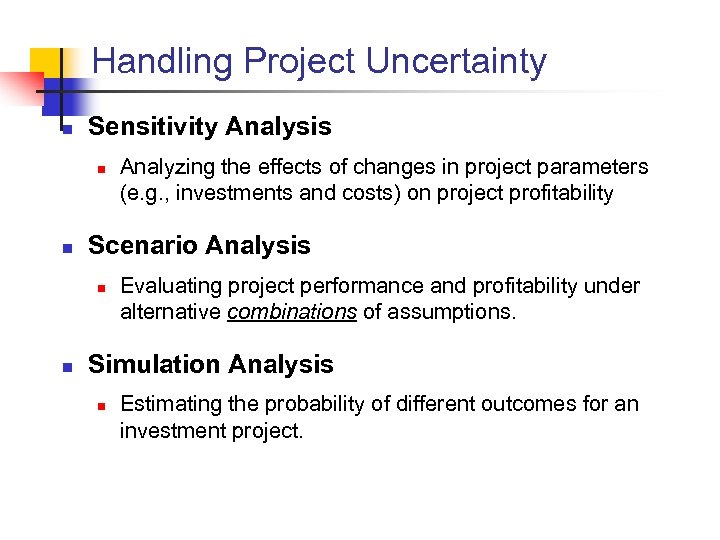Handling Project Uncertainty n Sensitivity Analysis n n Scenario Analysis n n Analyzing the effects of changes in project parameters (e. g. , investments and costs) on project profitability Evaluating project performance and profitability under alternative combinations of assumptions. Simulation Analysis n Estimating the probability of different outcomes for an investment project.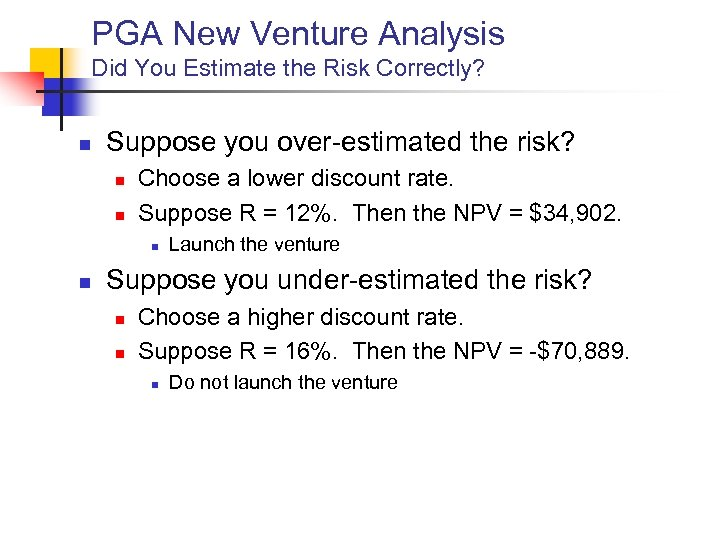PGA New Venture Analysis Did You Estimate the Risk Correctly? n Suppose you over-estimated the risk? n n Choose a lower discount rate. Suppose R = 12%. Then the NPV = \$34, 902. n n Launch the venture Suppose you under-estimated the risk? n n Choose a higher discount rate. Suppose R = 16%. Then the NPV = -\$70, 889. n Do not launch the venture